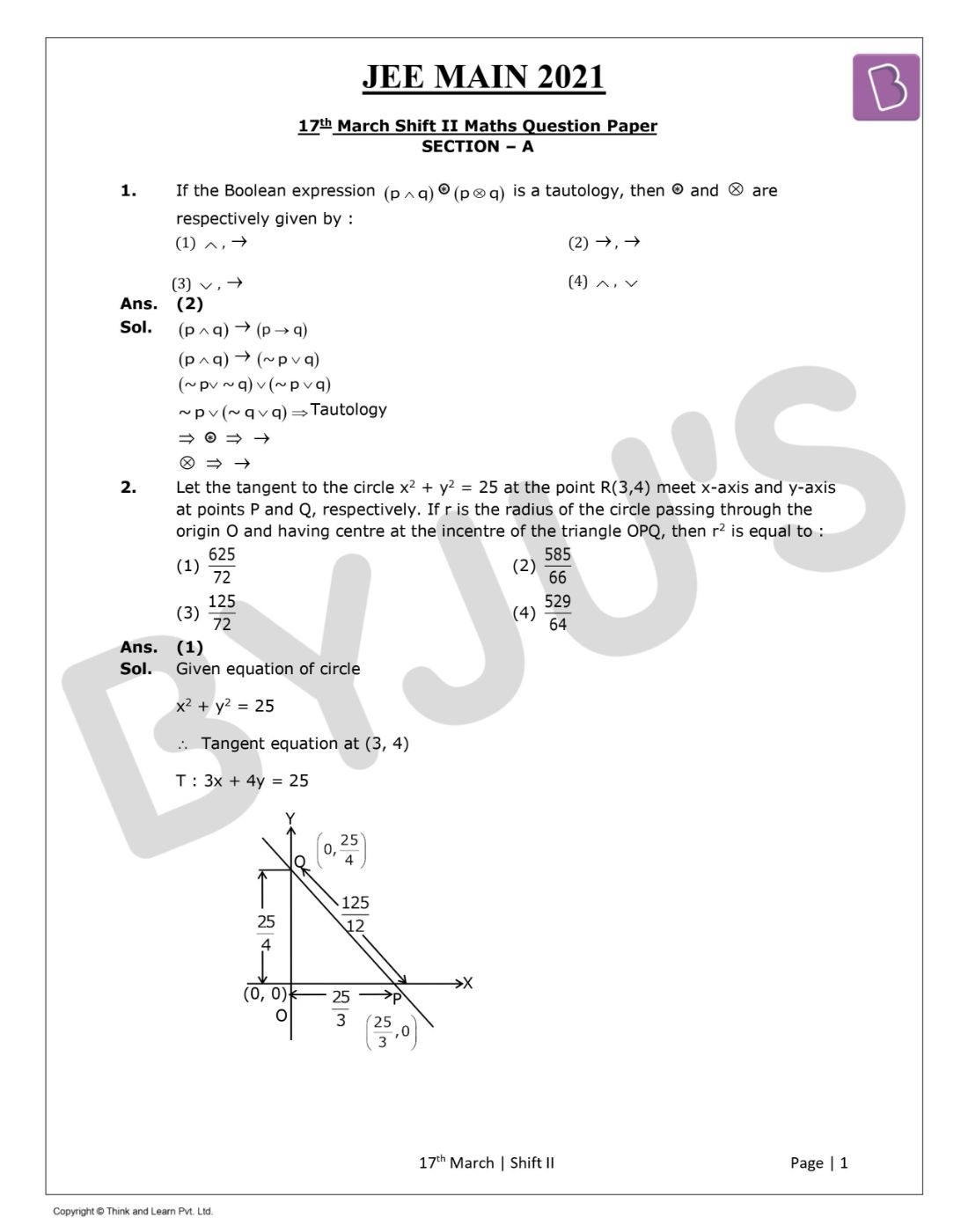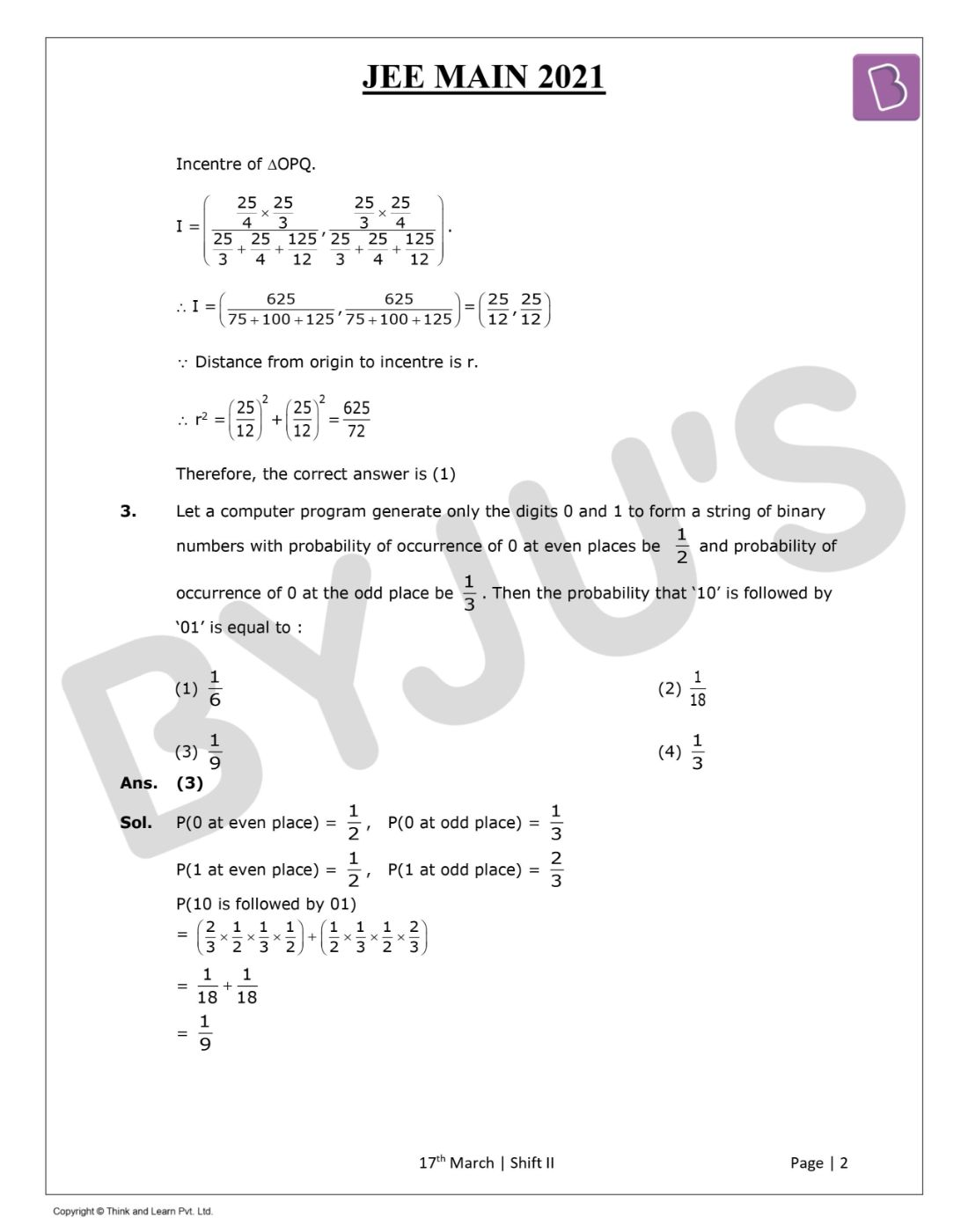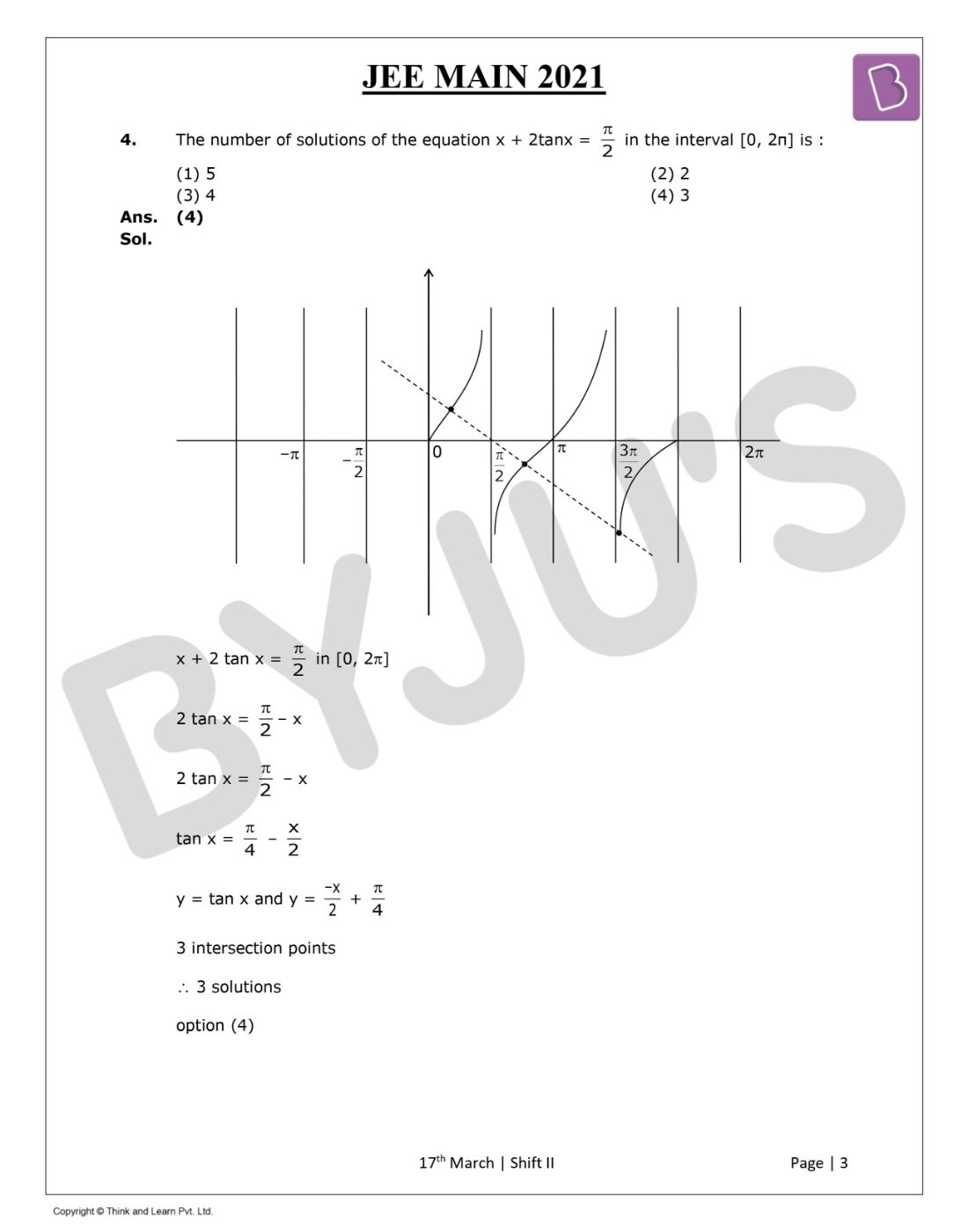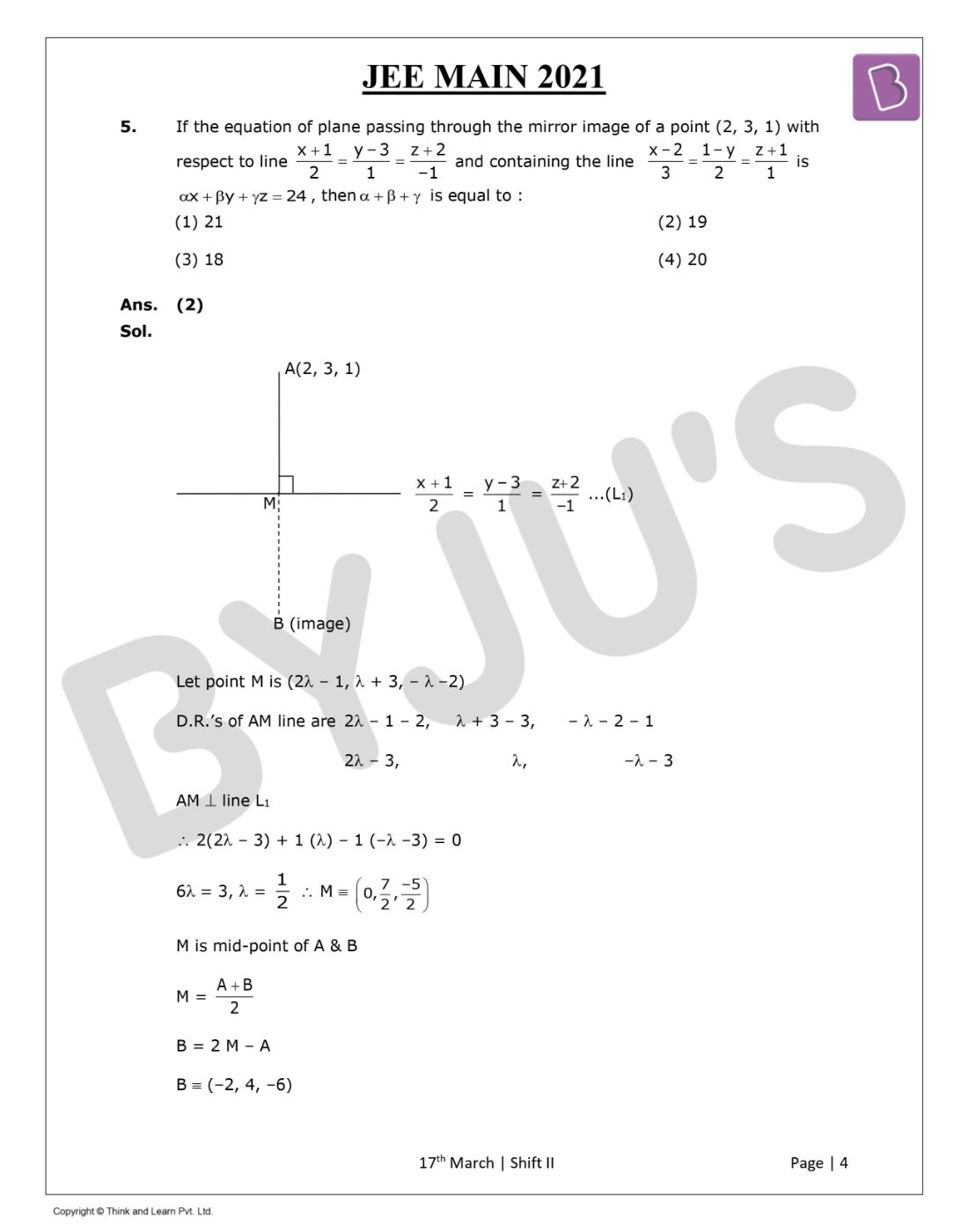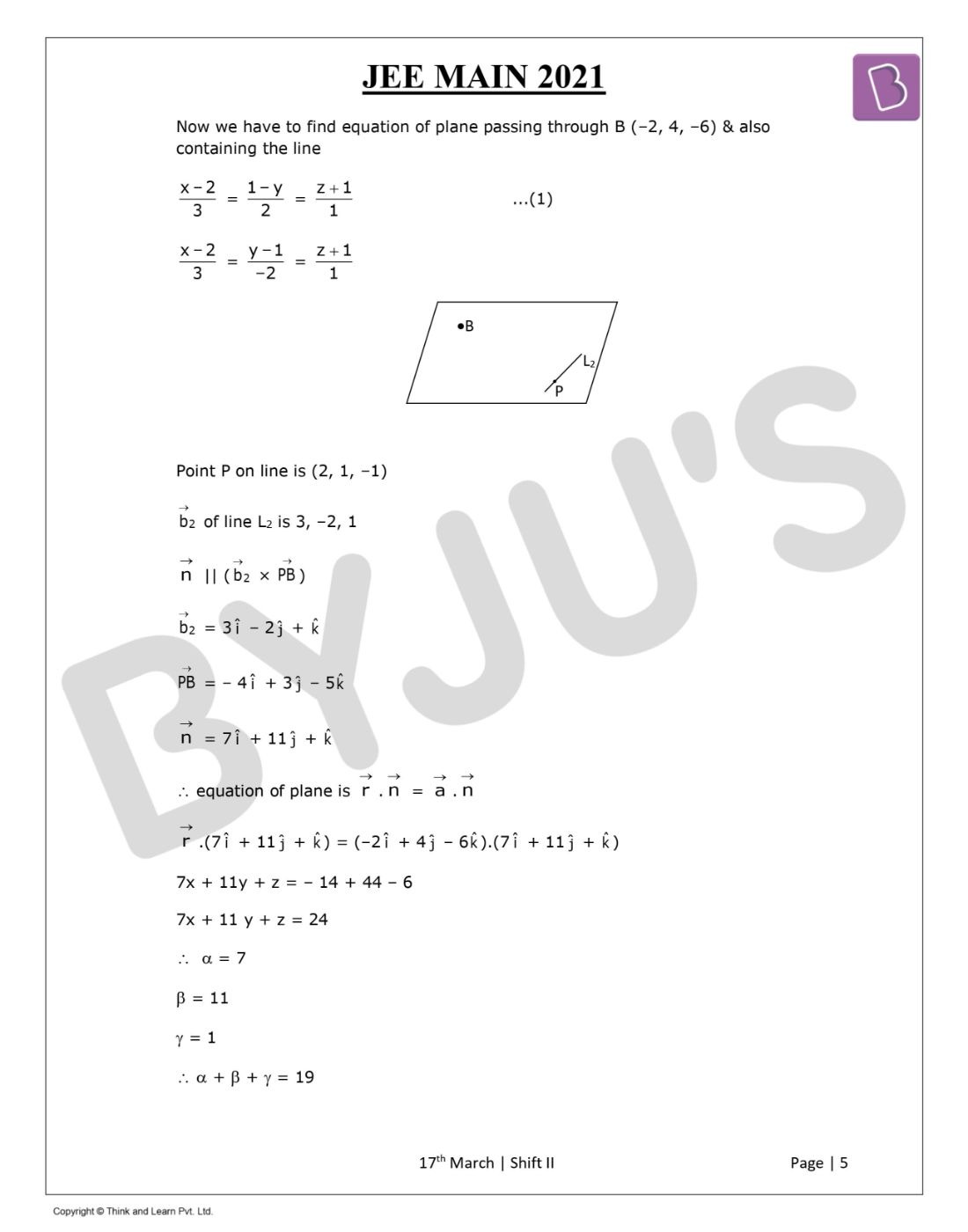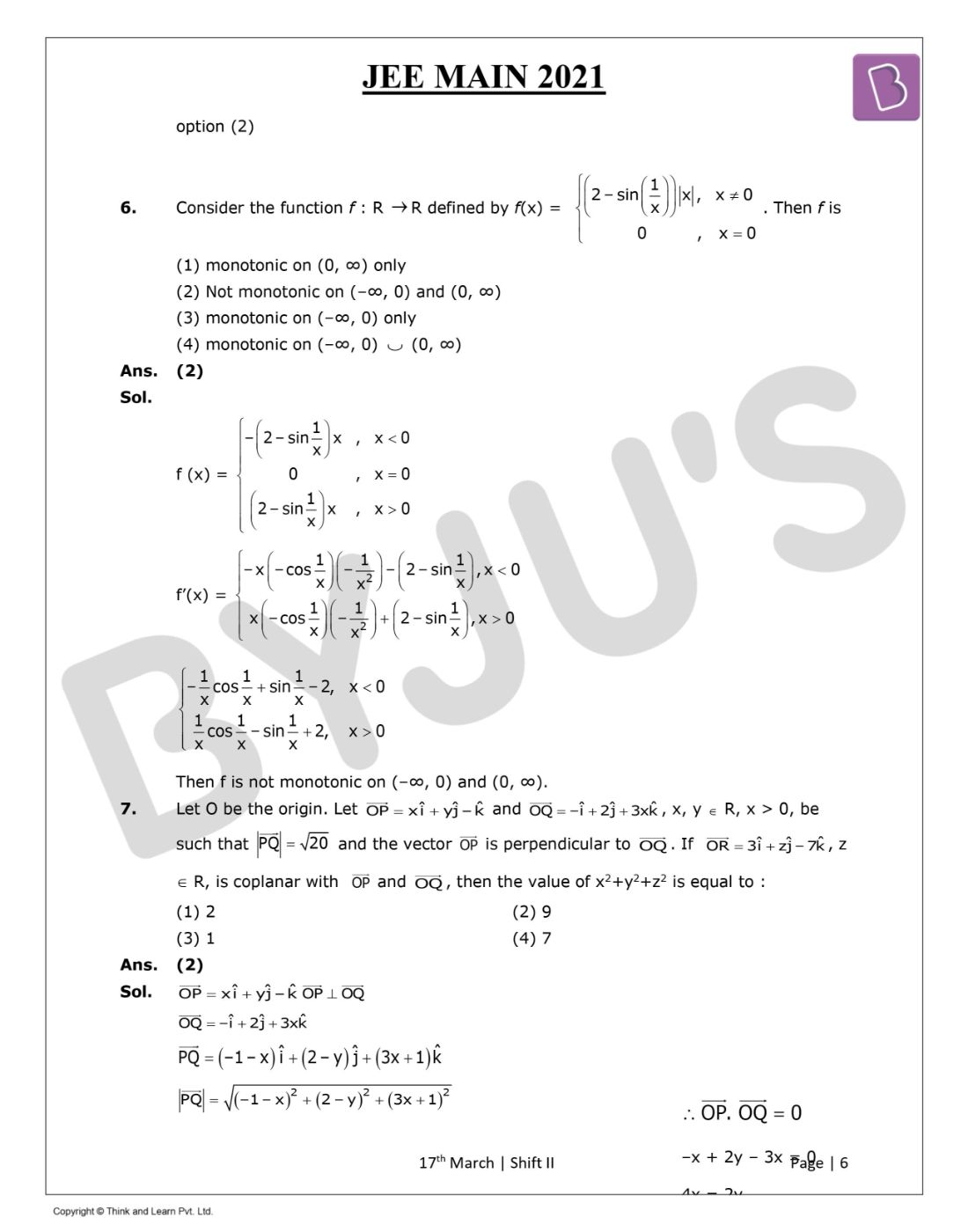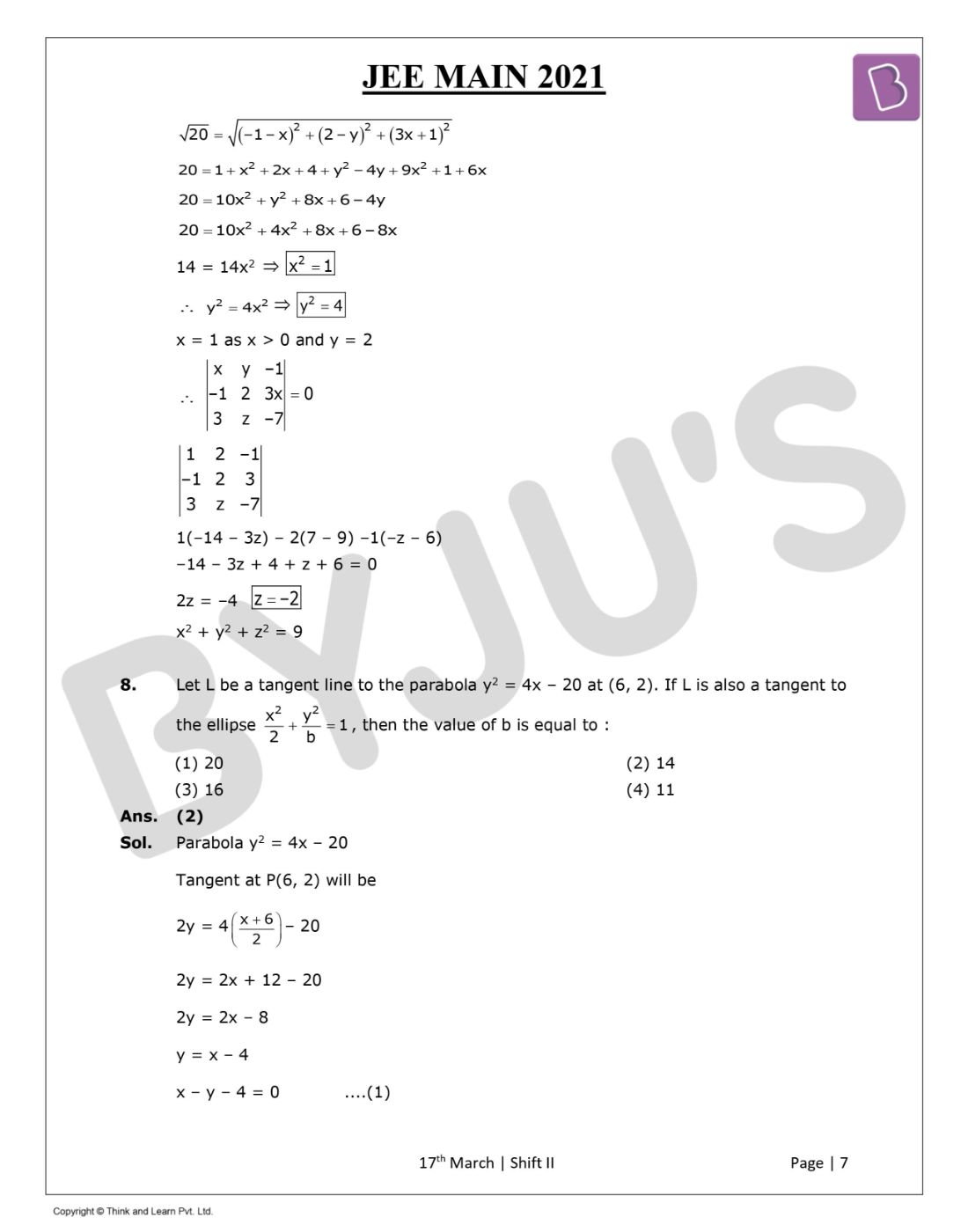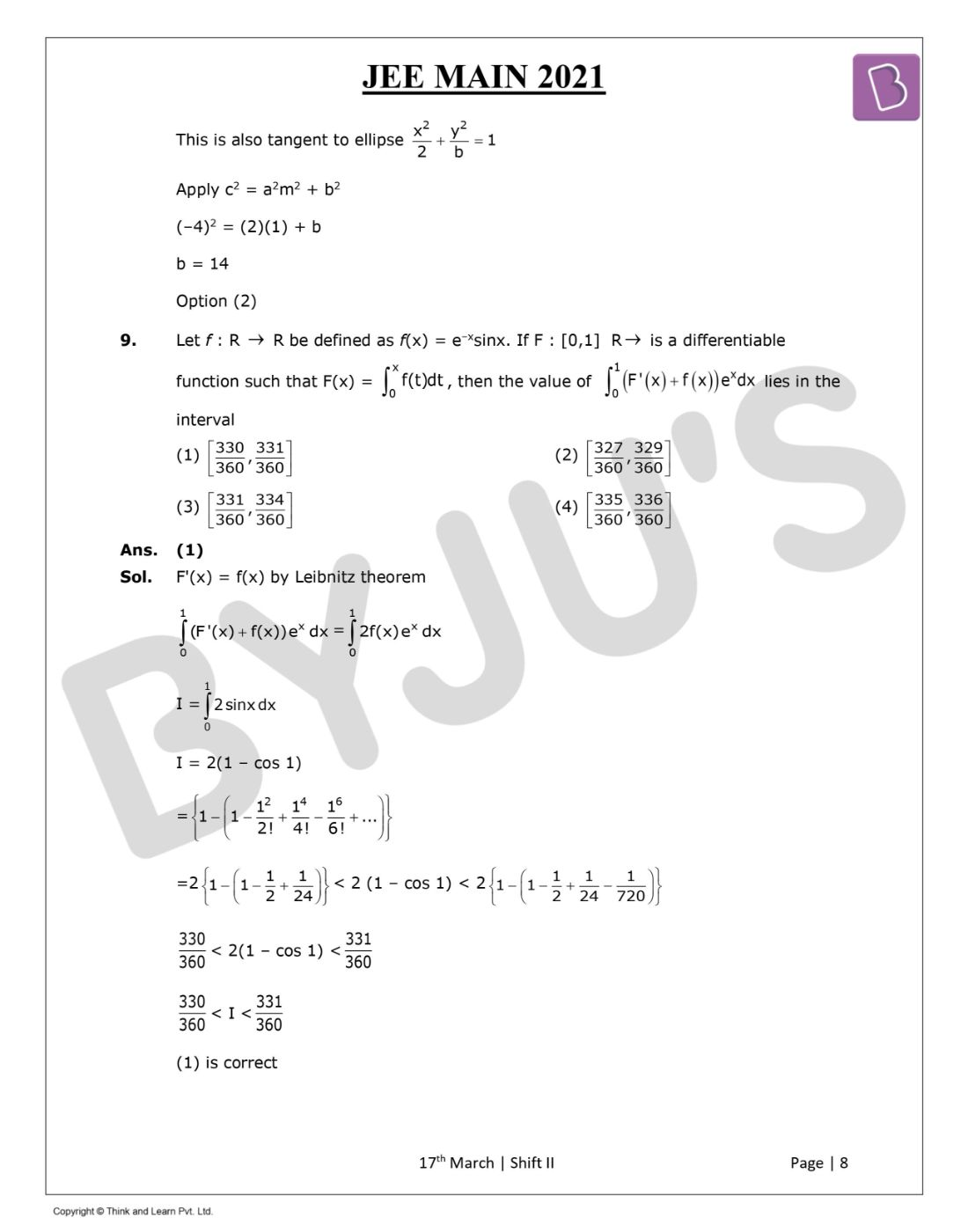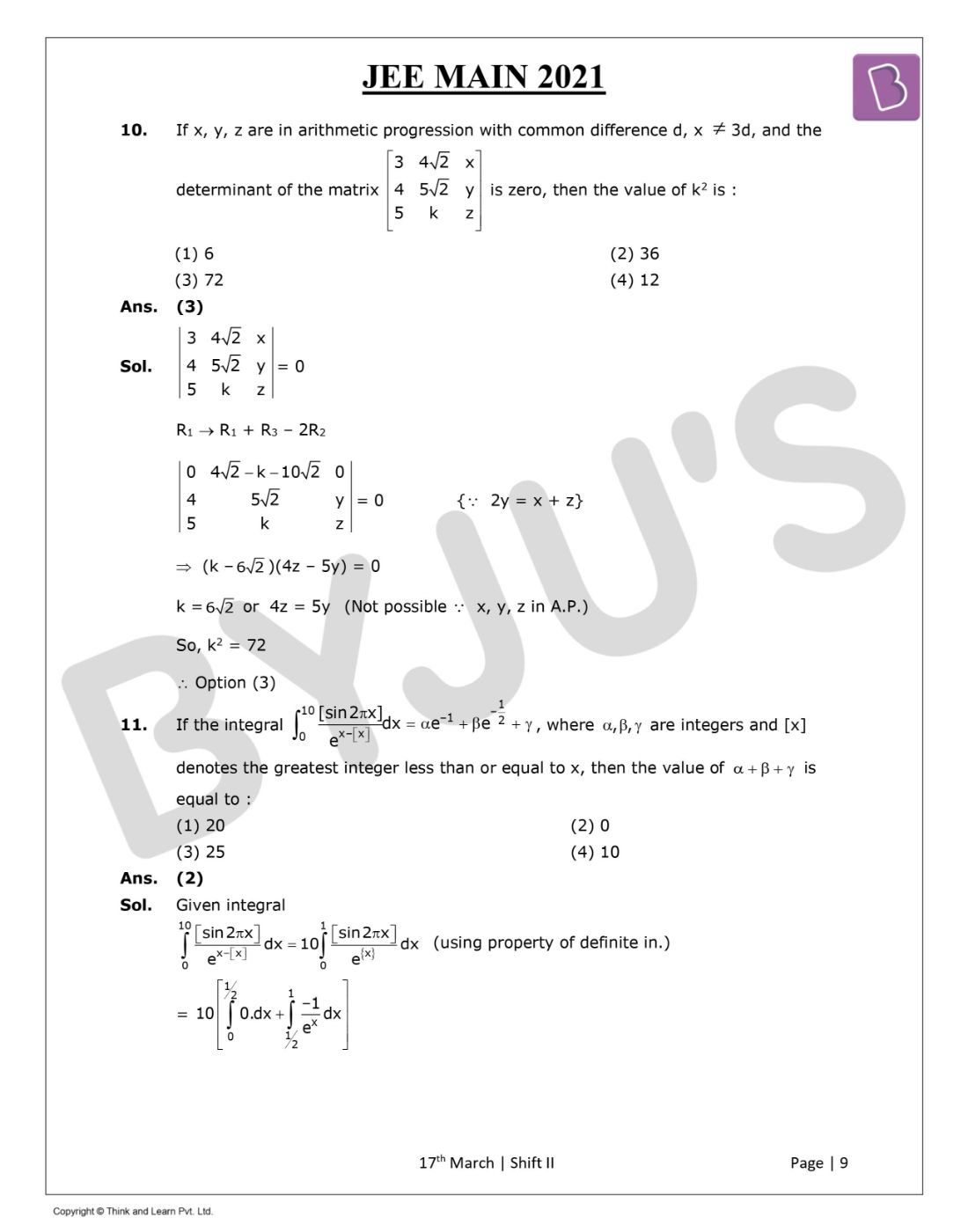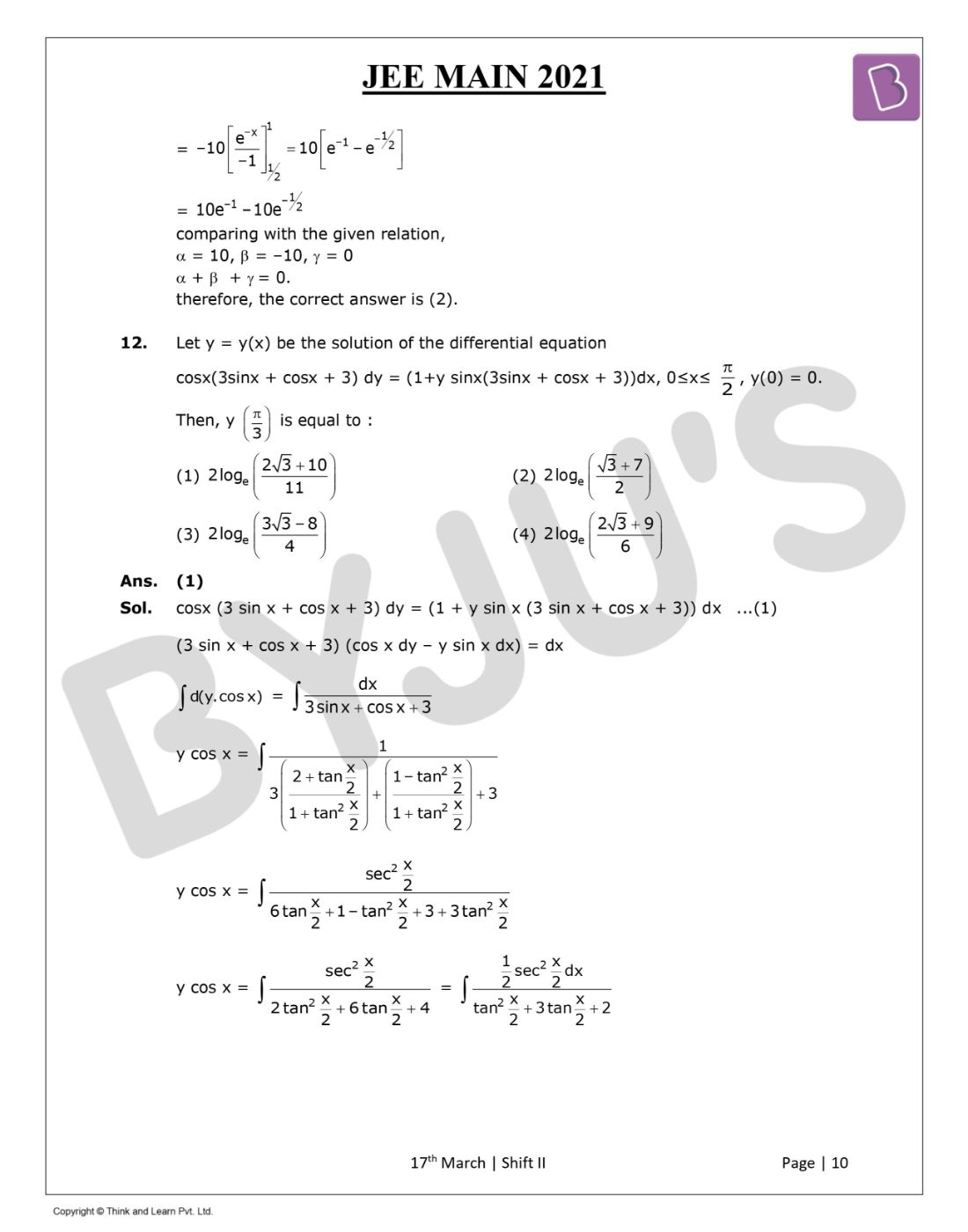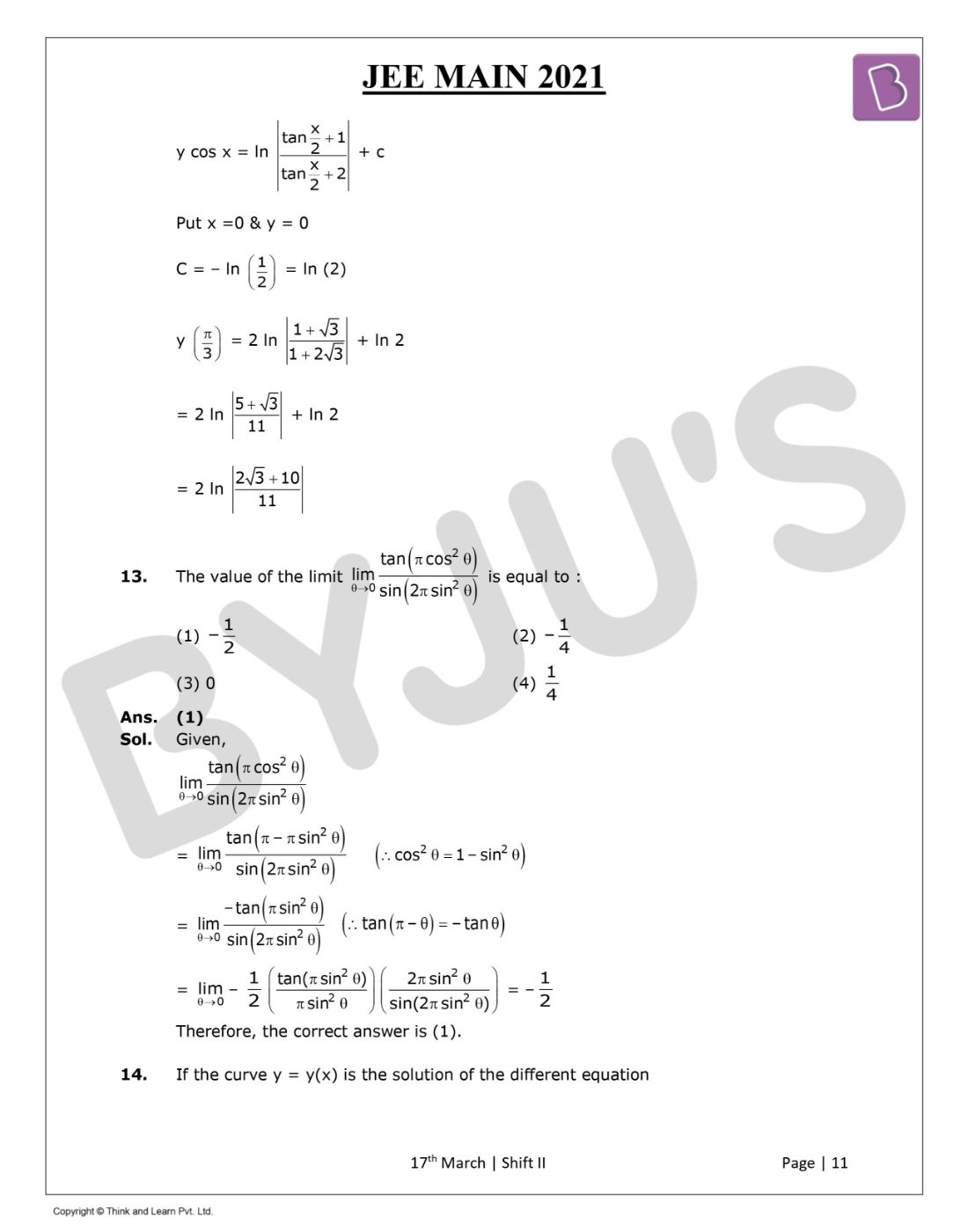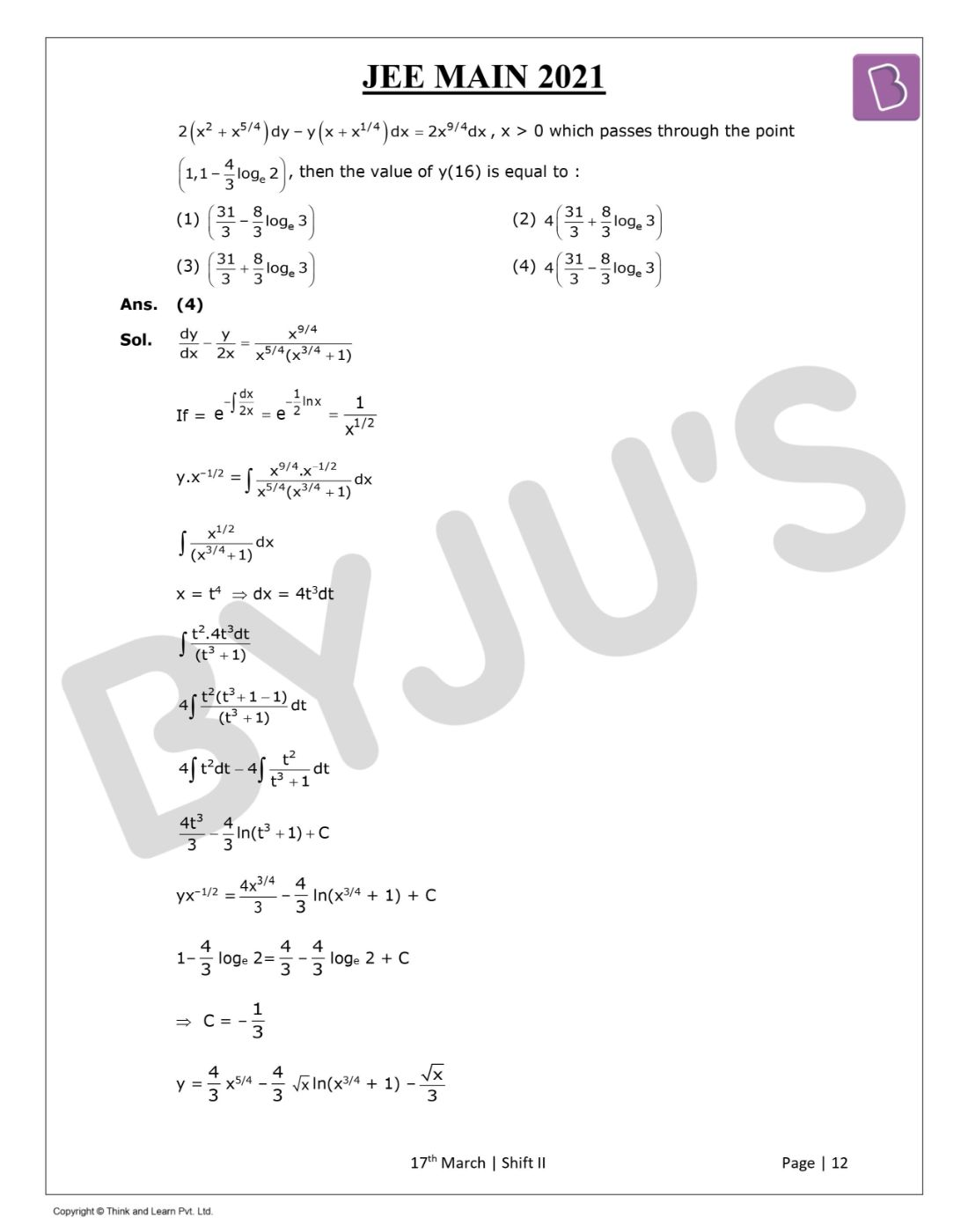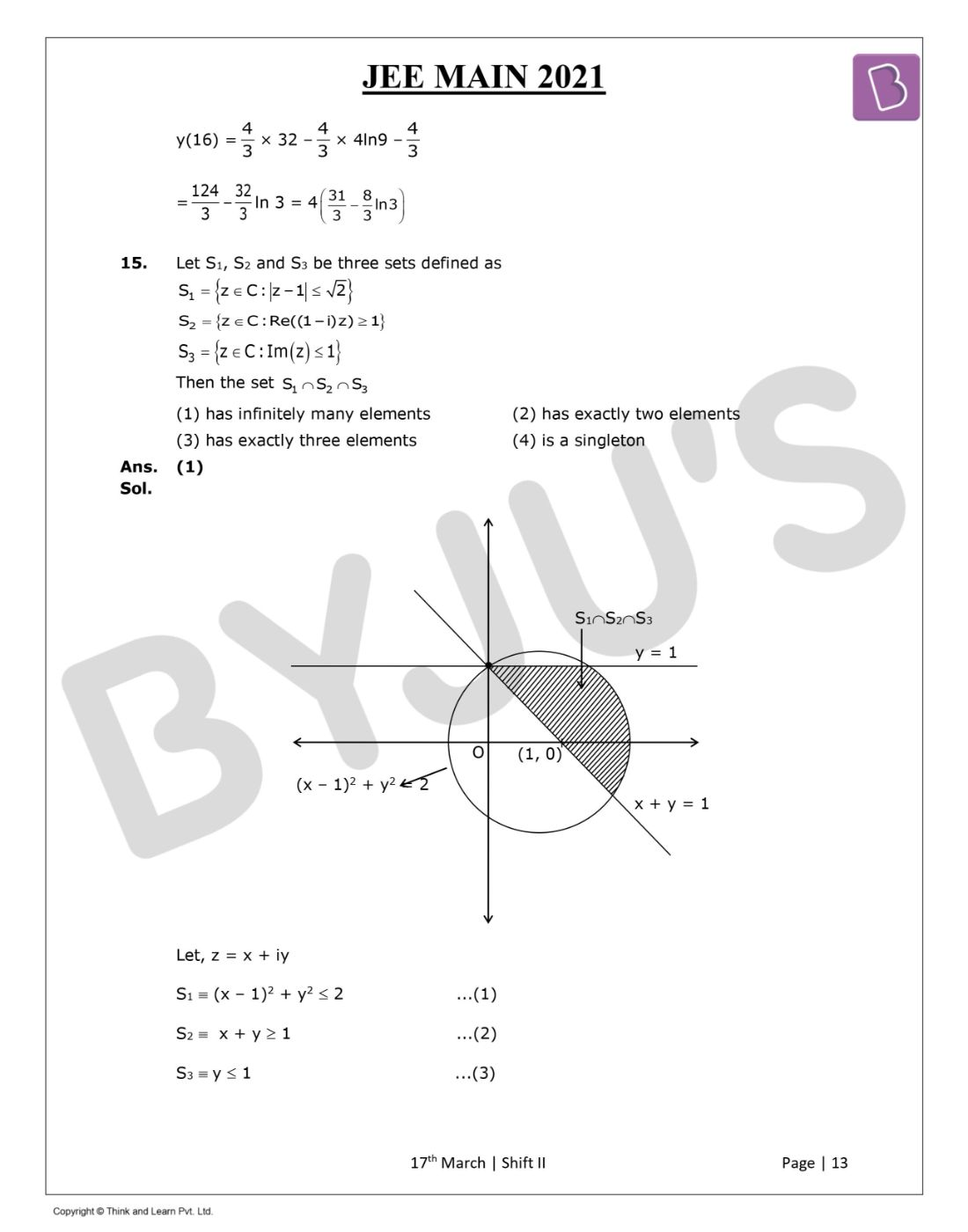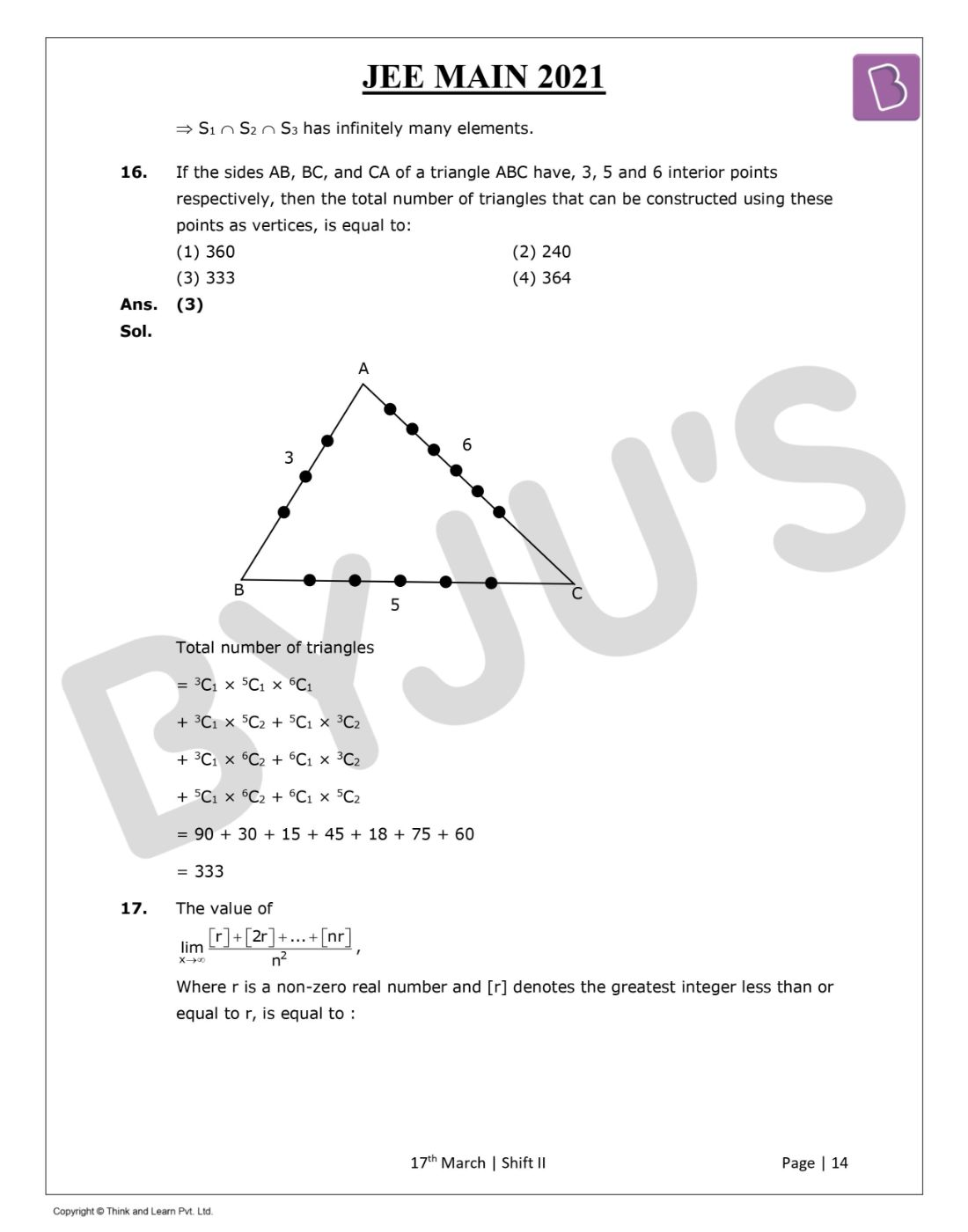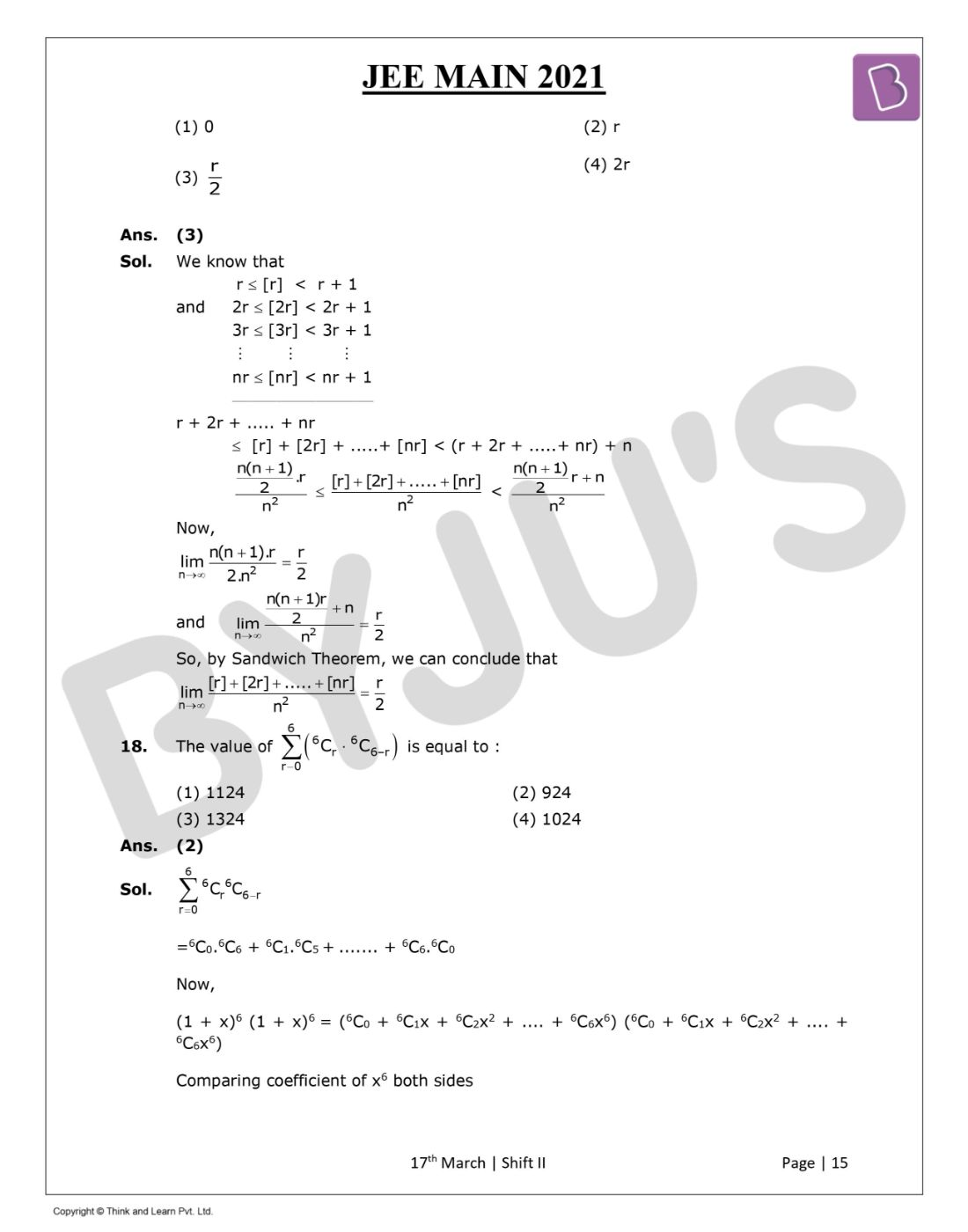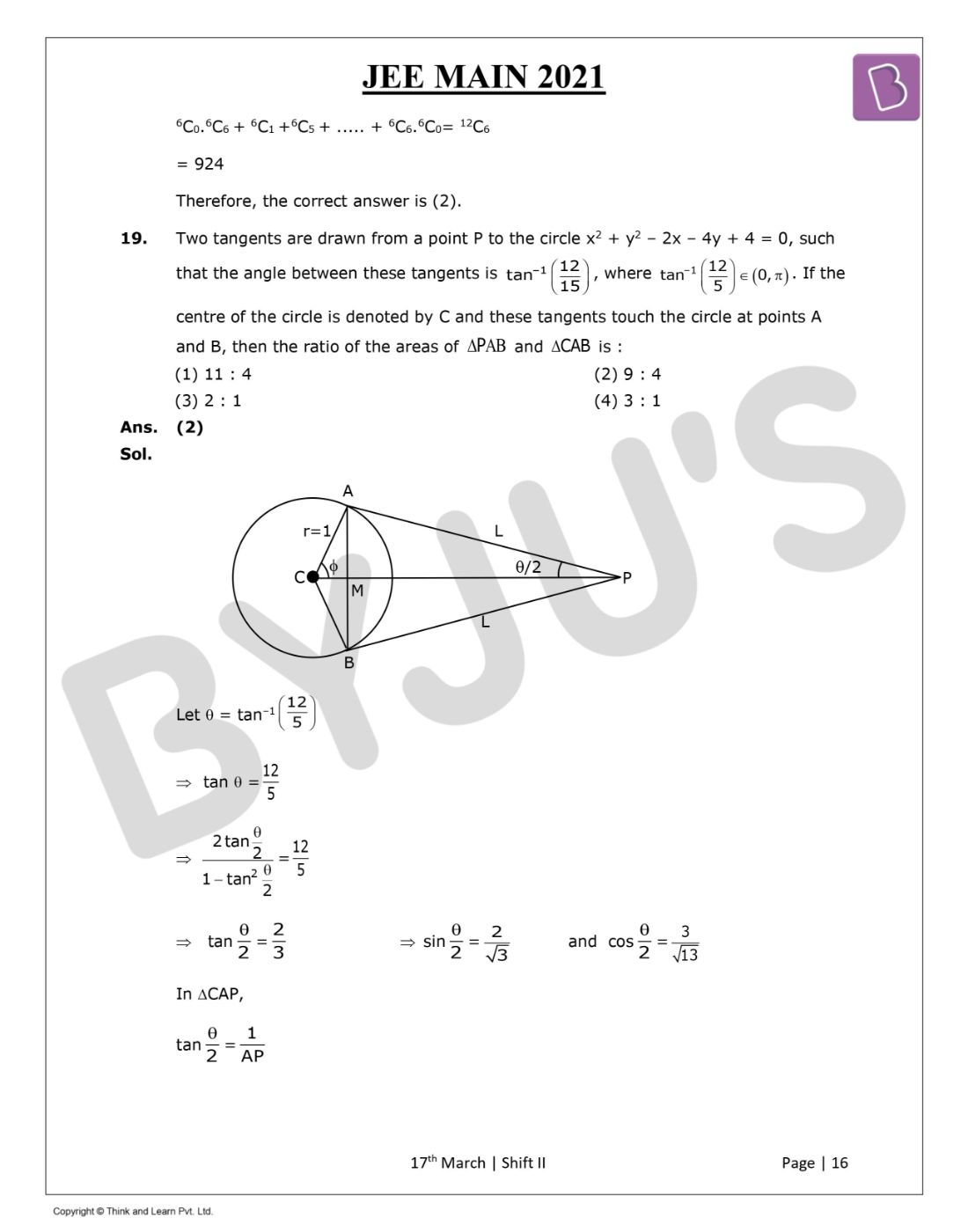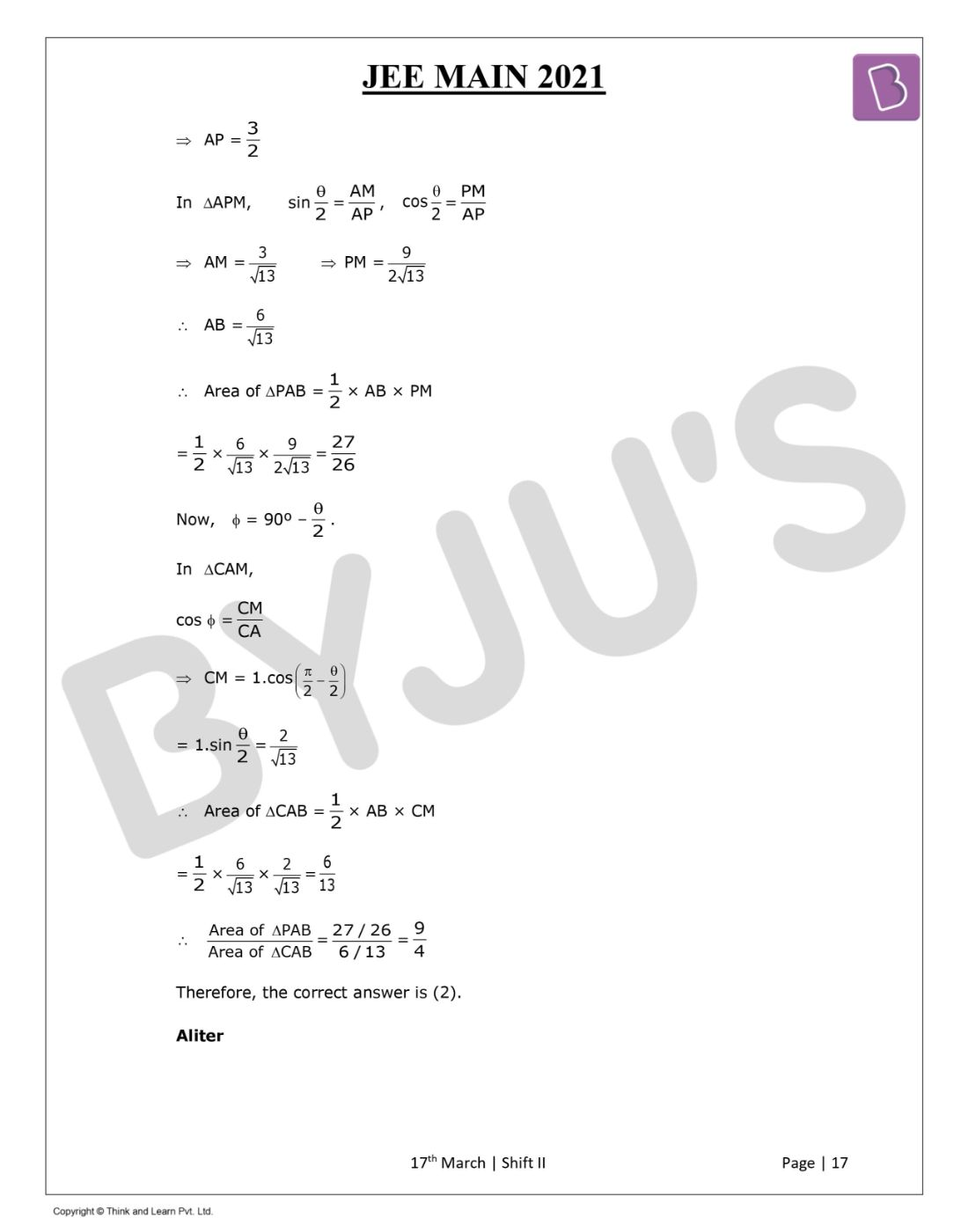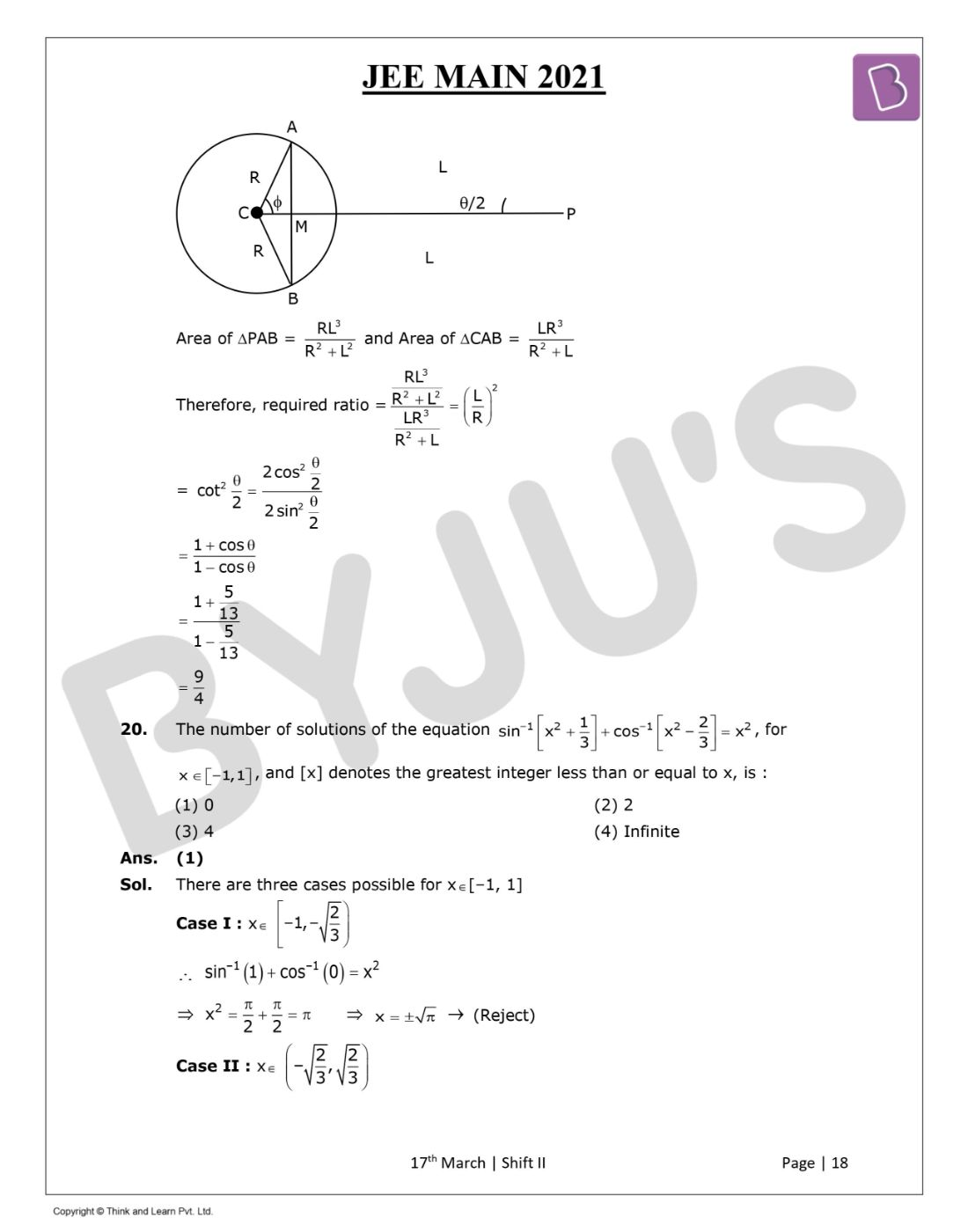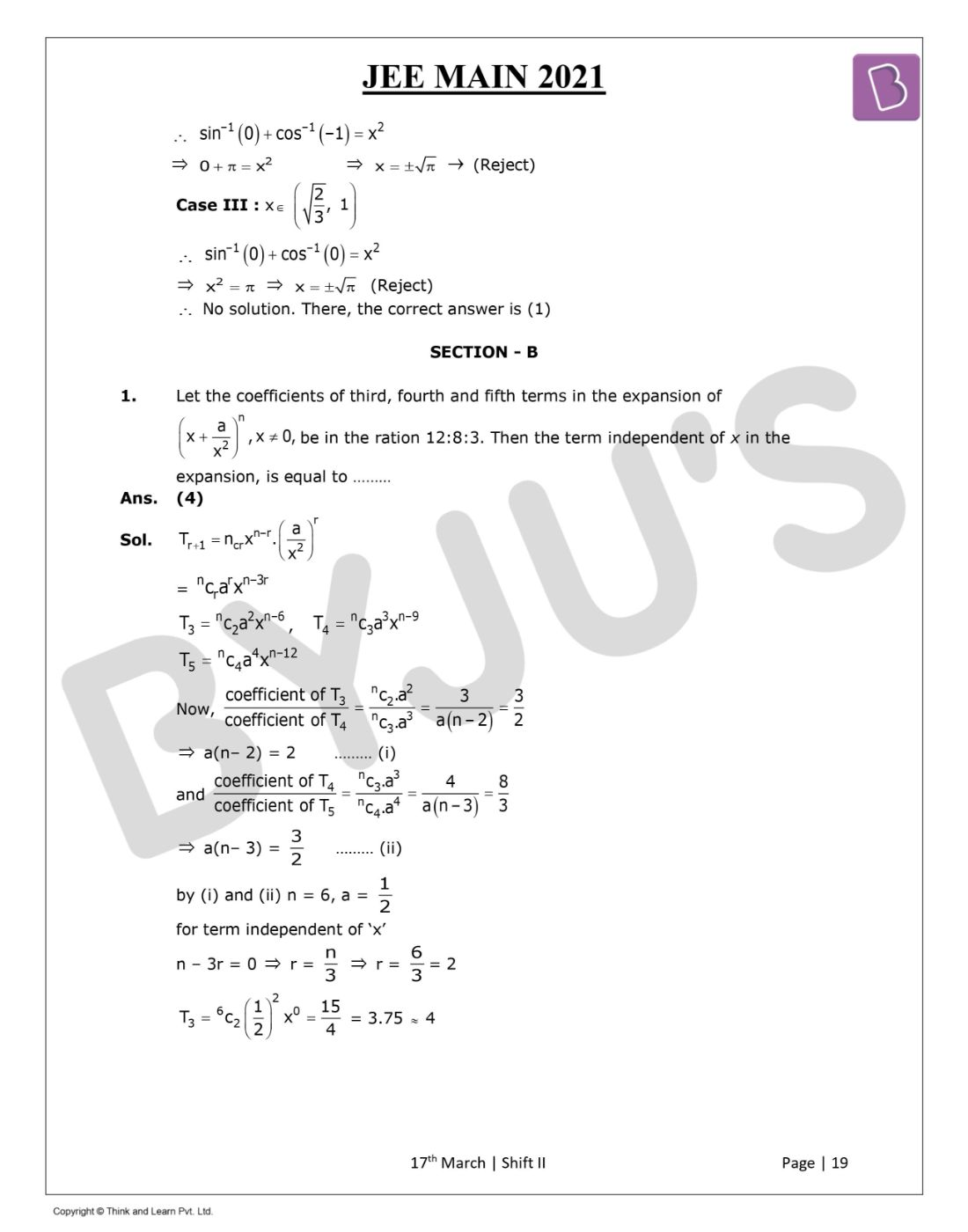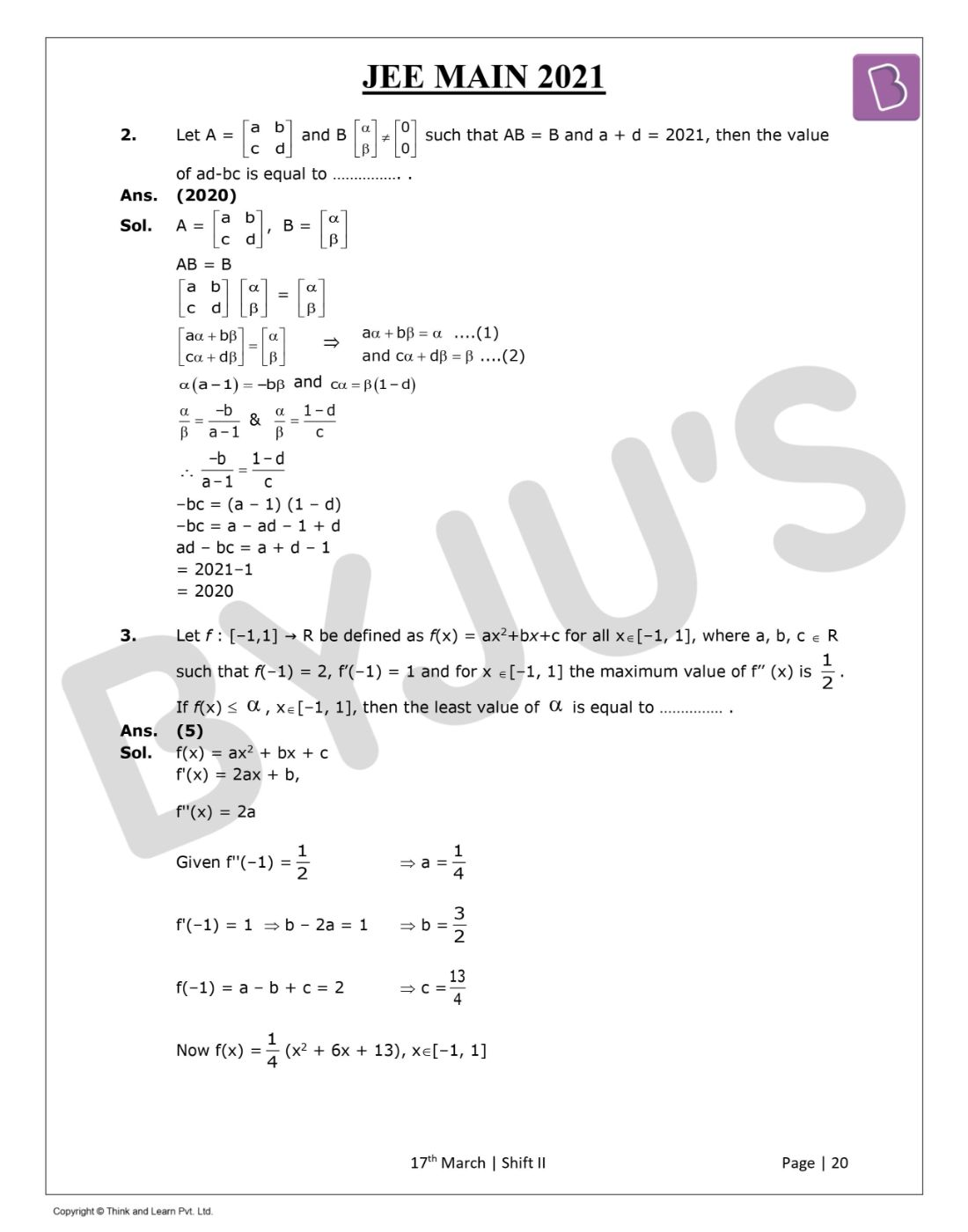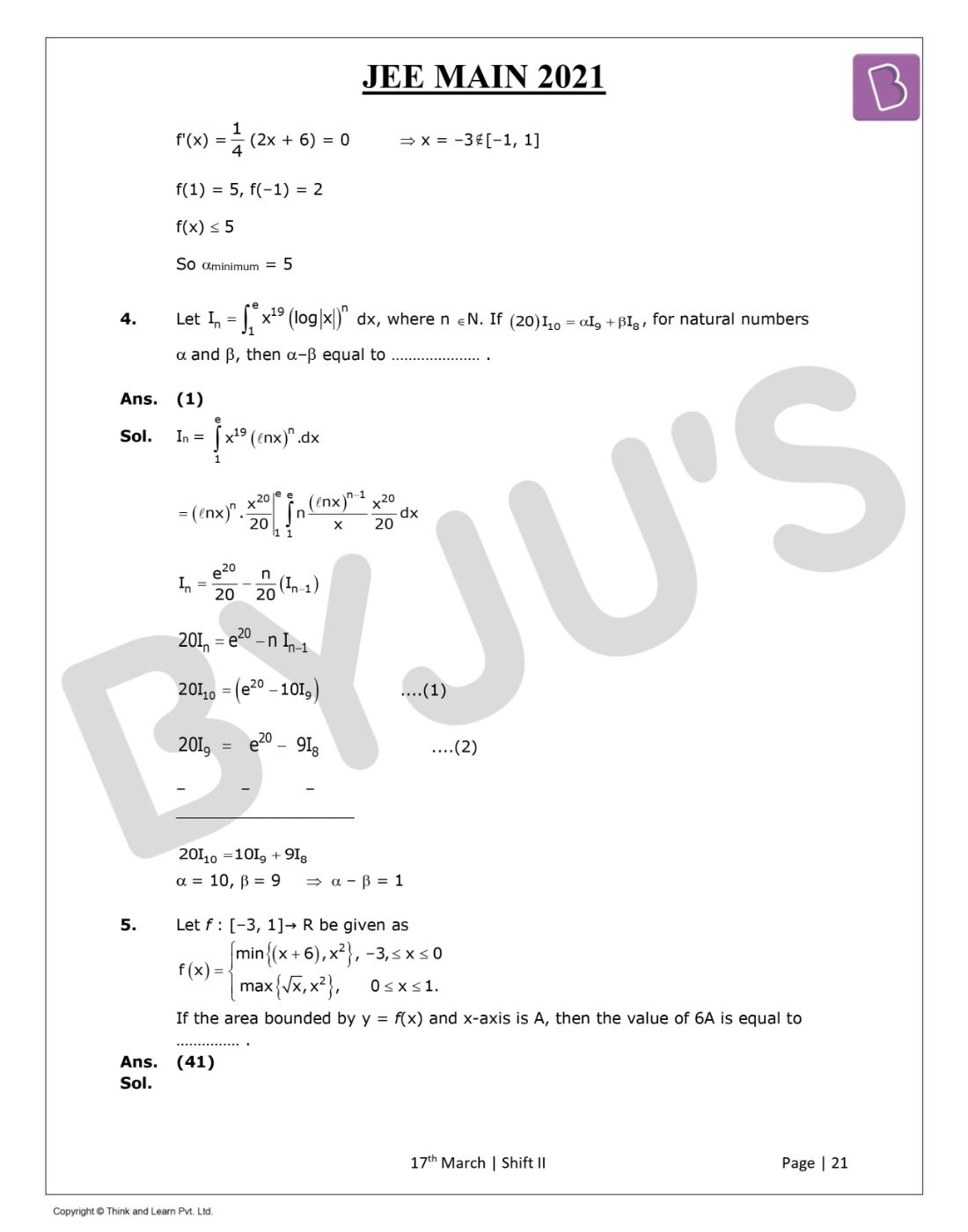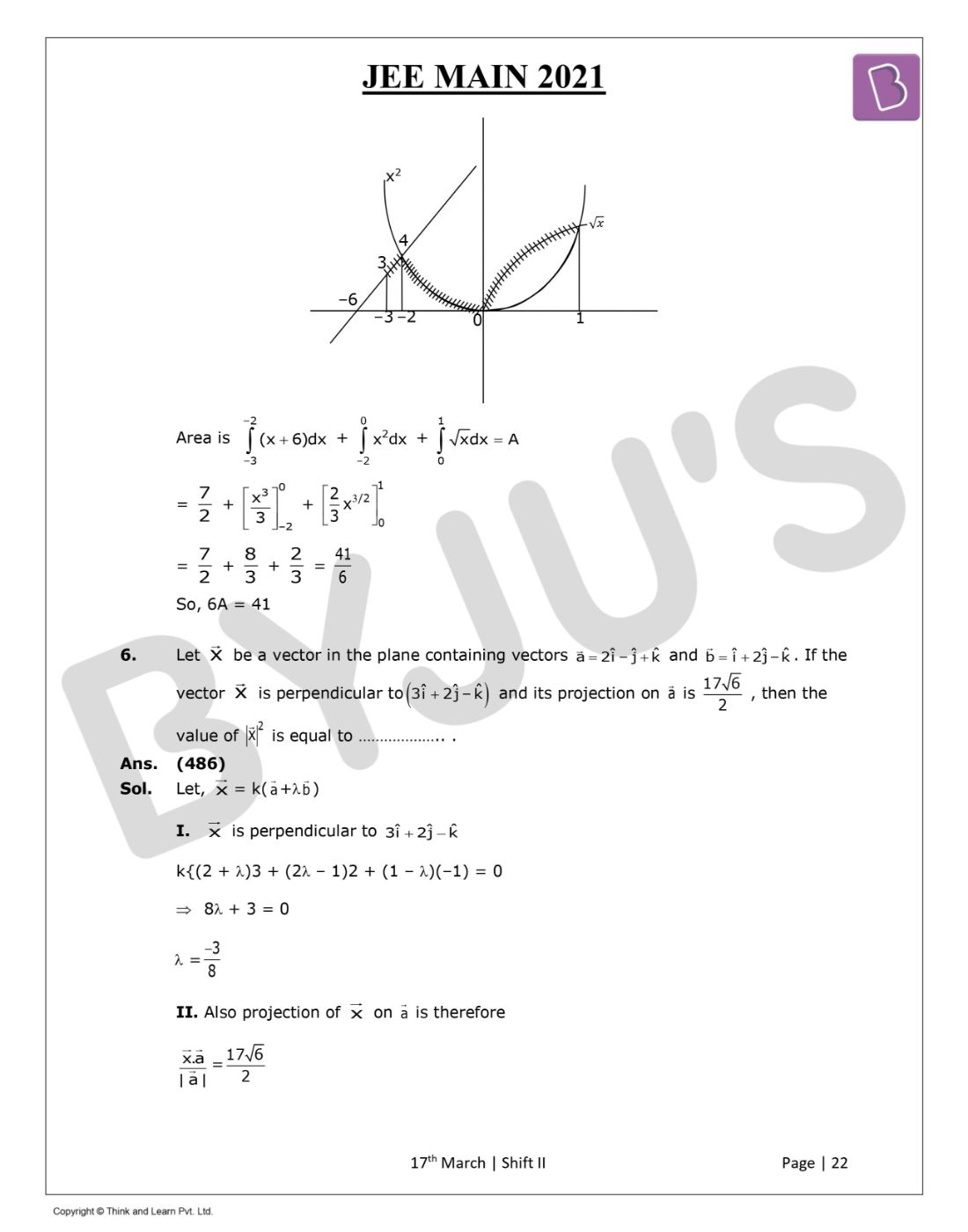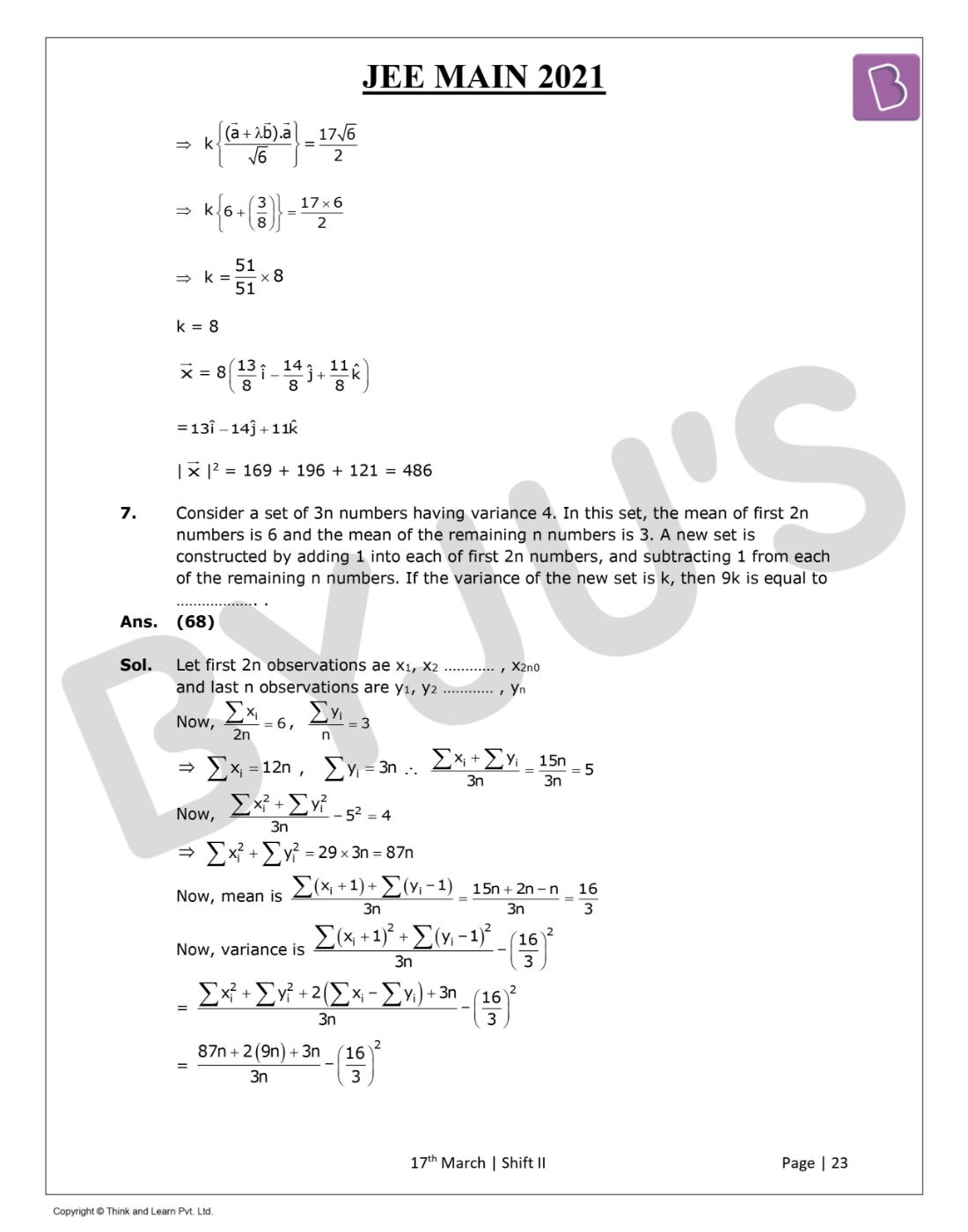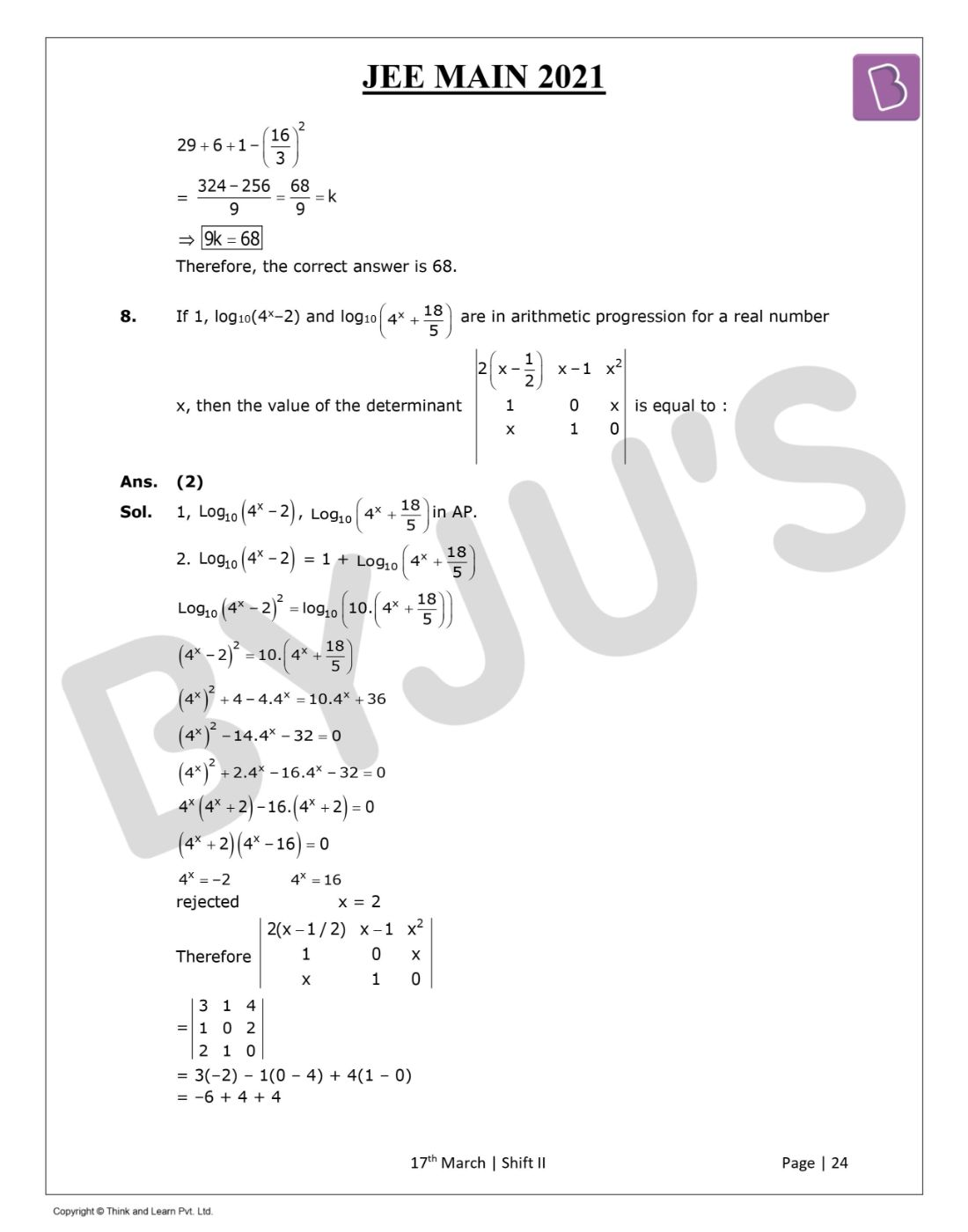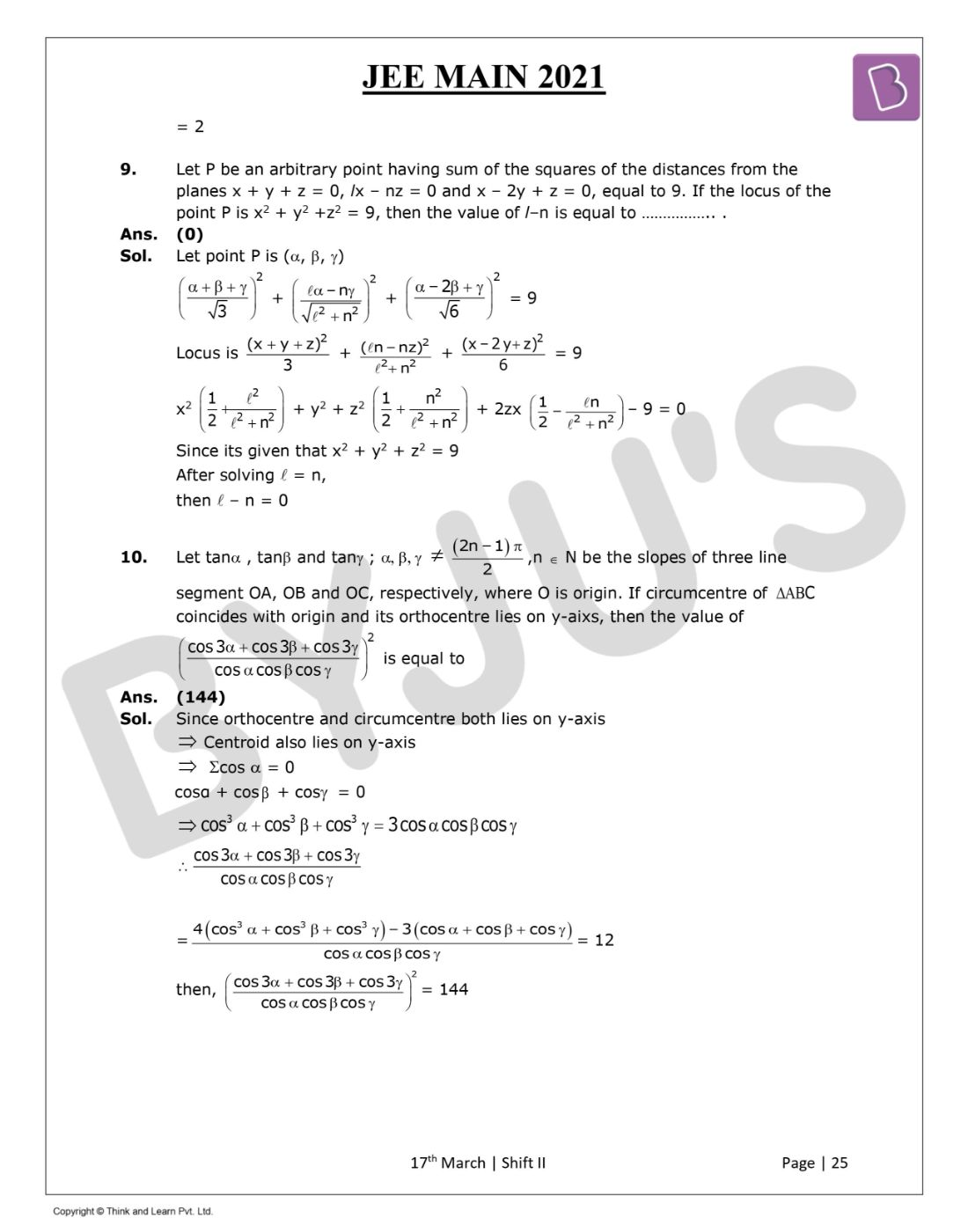Gauge your potential with Aakash BYJU'S JEE Main'23 Mock Test Gauge your potential with Aakash BYJU'S JEE Main'23 Mock Test

# JEE Main 2021 March 17 – Shift 2 Maths Question Paper with Solutions

JEE Main 2021 question paper for Maths (March 17 – Shift 2) is provided here along with solutions that have been prepared by our subject experts. Practicing these solutions will definitely help students to improve their problem-solving skills and thereby scoring higher marks. While referring to these JEE Main 2021 Maths questions and solutions students will be able to learn the problems quickly and easily. Students can also download the solved paper in a PDF format for offline practice.
JEE Main 2021 March 17th Shift 2 Maths Question Paper

Section – A

Question 1: If the Boolean expression (p ∧ q) ⍟ (p ⊗ q) is a tautology, then ⍟ and ⊗ are respectively given by :

a. ∧, →
b. →, →
c. ∨, →
d. ∧, ∨

(p ∧ q) → (p → q)

(p ∧ q) → (~ p ∨ q)

(~ p ∨ ~ q) ∨ (~ p ∨ q)

~ p ∨ (~ q ∨ q) ⇒ Tautology

⇒ →

⇒ →

Question 2: Let the tangent to the circle x2 + y2 = 25 at the point R (3, 4) meet the x-axis and y-axis at points P and Q, respectively. If r is the radius of the circle passing through the origin O and having a centre at the incentre of the triangle OPQ, then r2 is equal to:

a. 625 / 72
b. 585 / 66
c. 125 / 72
d. 529 / 64

Given equation of circle is x2 + y2 = 25.

Tangent equation at (3, 4) is given by T : 3x + 4y = 25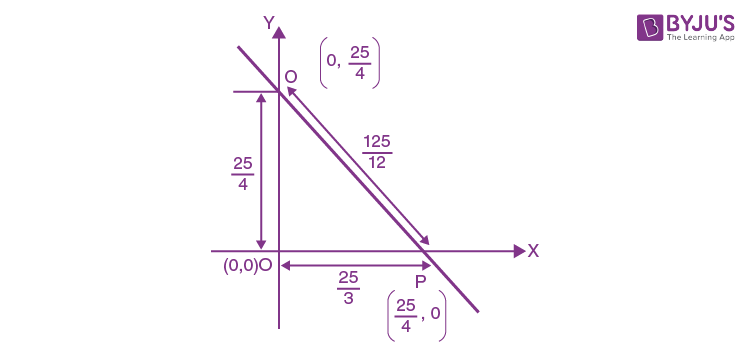Incentre of ΔOPQ is I = {[(25 / 4) * (25 / 3)] / [(25 / 3) + (25 / 4) + (125 / 12)], [(25 / 4) * (25 / 3)] / [(25 / 3) + (25 / 4) + (125 / 12)]

I = {[625 / (75 + 100 + 125)], [625 / (75 + 100 + 125)]} = (25 / 12, 25 / 12)

Distance from the origin to incentre is r.

∴ r2 = (25 / 12)2 + (25 / 12)2 = 625 / 72

Therefore, the correct answer is (1).

Question 3: Let a computer program generate only the digits 0 and 1 to form a string of binary numbers with a probability of occurrence of 0 at even places be 1 / 2 and probability of occurrence of 0 at the odd place be 1 / 3. Then the probability that ‘10’ is followed by ‘01’ is equal to :

a. 1 / 6
b. 1 / 18
c. 1 / 9
d. 1 / 3

P (0 at even place) = 1 / 2, P(0 at odd place) = 1 / 3

P (1 at even place) = 1 / 2, P(1 at odd place) = 2 / 3

P (10 is followed by 01) = [(2 / 3) * (1 / 2) * (1 / 3) * (1 / 2)] + [(1 / 2) * (1 / 3) * (1 / 2) * (2 / 3)]

= (1 / 18) + (1 / 18)

= 1 / 9

Question 4: The number of solutions of the equation x + 2 tanx = π / 2 in the interval [0, 2π] is :

a. 5
b. 2
c. 4
d. 3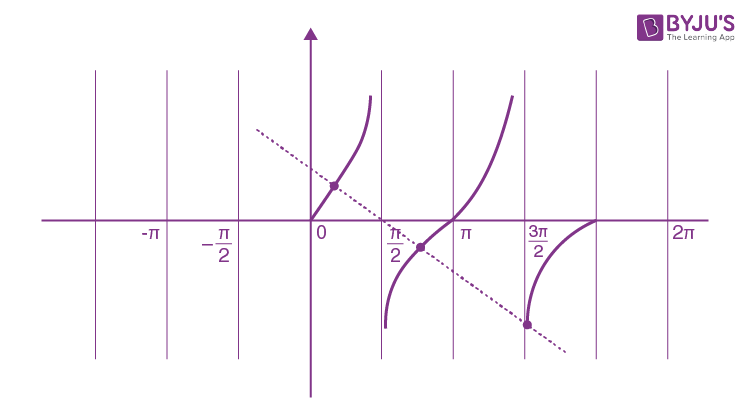x + 2 tan x = π / 2 in [0, 2π]

2 tan x = π / 2 – x

2 tan x = π / 2 – x

tan x = π / 4 – x / 2

y = tan x and y = (- x / 2) + π / 4

3 intersection points

∴ 3 solutions

Question 5: If the equation of the plane passing through the mirror image of a point (2, 3, 1) with respect to line (x + 1) / 2 = (y – 3) / 1 = (z + 2) / -1 and containing the line (x – 2) / 3 = (1 – y) / 2 = (z + 1) / 1 is ɑx + βy + 𝜸z = 24, then, then ɑ + β + 𝜸 is equal to:

a. 21
b. 19
c. 18
d. 20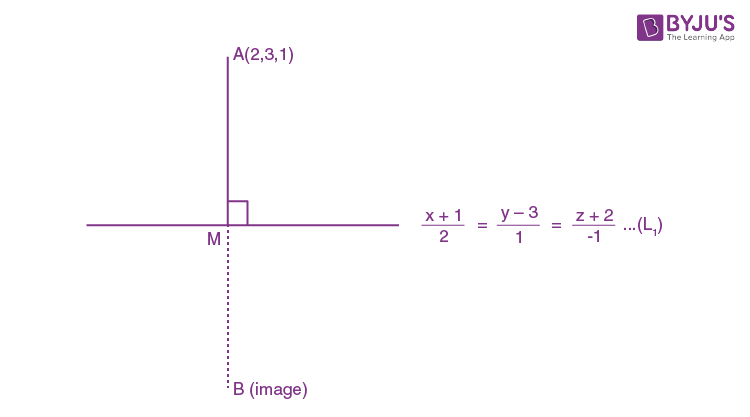Let point M is (2λ – 1, λ + 3, – λ – 2)

D.R.’s of AM line are: 2λ – 1 – 2, λ + 3 – 3, – λ – 2 – 1

2λ – 3, λ, –λ – 3

AM ⊥ line L1

∴ 2 (2λ – 3) + 1 (λ) – 1 (–λ – 3) = 0

6λ = 3, λ = 1 / 2

∴ M ≡ (0, 7 / 2, – 5 / 2)

M is mid-point of A & B

M = [A + B] / 2

B = 2 M – A

B ≡ (–2, 4, –6)

Now we have to find the equation of the plane passing through B (–2, 4, –6) & also containing the line

(x – 2) / 3 = (1 – y) / 2 = (z + 1) / 1 … (1)

(x – 2) / 3 = (y – 1) / – 2 = (z + 1) / 1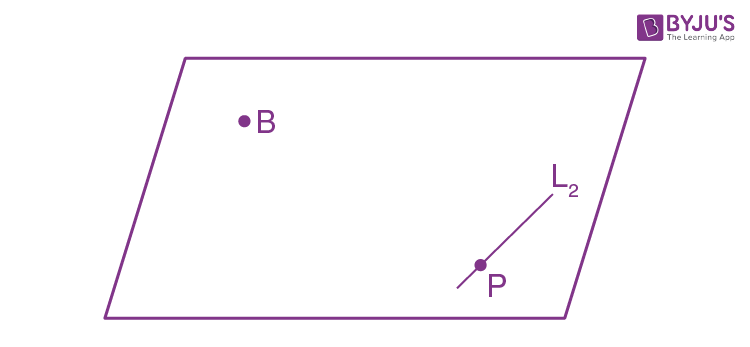Point P on line is (2, 1, –1)

b2 of line L2 is 3, –2, 1

n || (b2 x PB)

b2 = 3i – 2j + k

PB = – 4i + 3j – 5k

n = 7i + 11j + k

∴ equation of plane is r . n = a . n

r . (7i + 11j + k) = (–2i + 4j – 6k) . (7i + 11j + k)

7x + 11y + z = – 14 + 44 – 6

7x + 11 y + z = 24

∴ α = 7

β = 11

γ = 1

∴ α + β + γ = 19

Question 6: Consider the function f : R → R defined by f(x) =

$$\begin{array}{l}\left\{\begin{matrix} (2-sin(1/x)|x|), &x\neq 0 \\ 0&x=0 \end{matrix}\right.\end{array}$$
. Then f is

a. monotonic on (0, ∞) only
b. Not monotonic on (–∞, 0) and (0, ∞)
c. monotonic on (–∞, 0) only
d. monotonic on (–∞, 0) ⋃ (0, ∞)

$$\begin{array}{l}f(x)= \left\{\begin{matrix} -(2-sin(1/x)x), &x< 0 \\ 0&x=0 & \\ (2-sin(1/x)x)&x>0 \end{matrix}\right.\\ f'(x)=\left\{\begin{matrix} -x(-cos(1/x)(-1/x^2)-(2-sin(1/x))), &x<0 \\ x(-cos(1/x)(-1/x^2)+(2-sin(1/x))), &x>0 \end{matrix}\right.\\ \left\{\begin{matrix} -(1/x)cos(1/x)+sin(1/x)-2, &x<0 \\ (1/x)cos(1/x)-sin(1/x)+2, &x>0 \end{matrix}\right.\end{array}$$

Then f is not monotonic on (–∞, 0) and (0, ∞).

Question 7: Let O be the origin. Let OP = xi + yj – k and OQ = – i + 2j + 3xk, x, y ∈ R, x > 0, be such that |PQ| = √20 and the vector OP is perpendicular to OQ. If OR = 3i + zk – 7k, z ∈ R, is coplanar with OP and OQ, then the value of x2 + y2 + z2 is equal to:

a. 2
b. 9
c. 1
d. 7

OP = xi + yj – k

OP is perpendicular to OQ

OQ = – i + 2j + 3xk

PQ = (-1 – x) i + (2 – y) j + (3x + 1) k

|PQ| = √(- 1 – x)2 + (2 – y)2 + (3x + 1)2

OP . OQ = 0

– x + 2y – 3x = 0

4x – 2y=0

√20 = √(- 1 – x)2 + (2 – y)2 + (3x + 1)2

20 = 1 + x2 + 2x + 4 + y2 – 4y + 9x2 + 1 + 6x

20 = 10x2 + 4x2 + 8x + 6 – 8x

14 = 14x2

x2 = 1

y2 = 4x2

y2 = 4

x = 1 as x > 0 and y = 2

$$\begin{array}{l}\begin{vmatrix} x &y &-1 \\ -1&2 &3x \\ 3&z &-7 \end{vmatrix}=0\end{array}$$
$$\begin{array}{l}\begin{vmatrix} 1 &2 &-1 \\ -1&2 &3 \\ 3&z &-7 \end{vmatrix}=0\end{array}$$

1 (- 14 – 3z) – 2 (7 – 9) – 1 (- z – 6)=0

– 14 – 3z + 4 + z + 6 = 0

2z = – 4

z = – 2

x2 + y2 + z2 = 9

Question 8: Let L be a tangent line to the parabola y2 = 4x – 20 at (6, 2). If L is also a tangent to the ellipse (x2 / 2) + (y2 / b) = 1, then the value of b is equal to:

a. 20
b. 14
c. 16
d. 11

Parabola y2 = 4x – 20

Tangent at P (6, 2) will be

2y = 4 [(x + 6) / 2] – 20

2y = 2x + 12 – 20

2y = 2x – 8

y = x – 4

x – y – 4 = 0 ….(1)

This is also tangent to ellipse (x2 / 2) + (y2 / b) = 1

Apply c2 = a2m2 + b2

(–4)2 = (2)(1) + b

b = 14

Question 9: Let f : R → R be defined as f (x) = e–x sinx. If F : [0,1] R→ is a differentiable function such that F (x) = ∫0x f (t) dt, then the value of ∫01 [F’ (x) + f (x)] ex dx lies in the interval

a. [330 / 360, 331 / 360]
b. [327 / 360, 329 / 360]
c. [331 / 360, 334 / 360]
d. [335 / 360, 336 / 360]

F’ (x) = f (x) by Leibnitz theorem

01 [F’ (x) + f (x)] ex dx = ∫01 2 f (x) ex dx

I = ∫01 2 sinx dx

I = 2 (1 – cos 1)

= {1 – [1 – (12 / 2!) + (14 / 4!) – (16 / 6!) + ……]}

= 2 {1 – [1 – (1 / 2) + (1 / 24)]} < 2 (1 – cos 1) < 2 {1 – [1 – (1 / 2) + (1 / 24) – (1 / 720)]}

330 / 360 < 2(1 – cos 1) < 331 / 360

330 / 360 < I < 331 / 360

Choice (a) is correct.

Question 10: If x, y, z are in arithmetic progression with common difference d, x ≠ 3d, and the determinant of the matrix

$$\begin{array}{l}\begin{bmatrix} 3 &4\sqrt{2} &x \\ 4&5\sqrt{2} &y \\ 5&k &z \end{bmatrix}=0\\\end{array}$$
is zero, then the value of k2 is:

a. 6
b. 36
c. 72
d. 12

$$\begin{array}{l}\\\begin{bmatrix} 3 &4\sqrt{2} &x \\ 4&5\sqrt{2} &y \\ 5&k &z \end{bmatrix}=0\\ R_{1}\rightarrow R_{1}+R_{3}-2R_{2}\\ \begin{bmatrix} 0 &4\sqrt{2}+k-10\sqrt{2} &0 \\ 4&5\sqrt{2} &y \\ 5&k &z \end{bmatrix}=0\\ -(k-6\sqrt{2})(4z-5y)=0\\ k=6\sqrt{2}\\ 4z=5y(not\ possible)\\ k^{2}=72\end{array}$$

Question 11: If the integral ∫010 [sin 2πx] / ex-[x] dx = ɑe-1 + βe + 𝛾, where ɑ, β, 𝞬 are integers and [x] denotes the greatest integer less than or equal to x, then the value of ɑ + β + 𝞬 is equal to:

a. 20
b. 0
c. 25
d. 10

Given integral ∫010 [sin 2πx] / ex-[x] dx = 10 ∫01 [sin 2πx] / e[x] dx (using the property of definite integral]

= 10 [∫01/2 0 . dx + ∫1/21 – 1 / ex dx]

= – 10 [e-x / – 1]1/21

= 10 [e-1 – e]

= 10 e-1 – 10e-1/2

Comparing with the given relation,

α = 10, β = –10, γ = 0

α + β + γ = 0.

Therefore, the correct answer is (2).

Question 12: Let y = y (x) be the solution of the differential equation cosx (3 sinx + cosx + 3) dy = (1 + y sinx (3 sinx + cosx + 3)) dx, 0 ≤ x ≤ π / 2, y(0) = 0. Then, y (π / 3) is equal to :

a. 2 loge [(2√3 + 10) / 11]
b. 2 loge [(√3 + 7) / 2]
c. 2 loge [(3√3 – 8)] / 4
d. 2 loge [(2√3 + 9) / 6]

cosx (3 sin x + cos x + 3) dy = (1 + y sin x (3 sin x + cos x + 3)) dx …(1)

(3 sin x + cos x + 3) (cos x dy – y sin x dx) = dx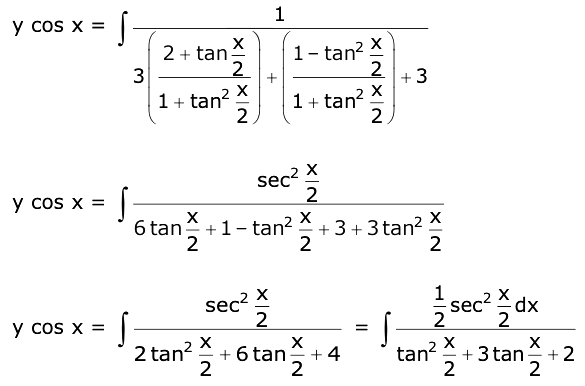∫ d (y . cosx) = ∫ dx / [3 sinx + cosx + 3]

y cos x = ln |(tan (x / 2) + 1) / [tan (x / 2) + 2]| + c

Put x = 0 & y = 0

C = – ln (1 / 2) = ln (2)

y (π / 3) = 2 ln |(1 + √3) / (1 + 2√3)| + ln 2

= 2 ln |(5 + √3) / 11| + ln 2

= 2 ln |(2 √3 + 10) / 11|

Question 13: The value of the limit limθ→0 [tan (π cos2 θ)] / [sin (2π sin2 θ)] is equal to:

a. – 1 / 2
b. – 1 / 4
c. 0
d. 1 / 4

Given,

limθ→0 [tan (π cos2 θ)] / [sin (2π sin2 θ)]

= limθ→0 [tan (π – π sin2 θ)] / [sin (2π sin2 θ)]

= limθ→0 [- tan (π sin2 θ)] / [sin (2π sin2 θ)]

= limθ→0 (-1 / 2) {[tan (π sin2 θ)] / [π sin2 θ]} * {[2π sin2 θ] / [sin (2π sin2 θ)]} = – 1 / 2

Therefore, the correct answer is (1).

Question 14: If the curve y = y(x) is the solution of the differential equation 2 (x2 + x5/4) dy – y (x + x1/4) dx = 2x9/4 dx, x > 0 which passes through the point [1, 1 – (4 / 3) loge 2] , then the value of y (16) is equal to:

a. [(31 / 3) – (8 / 3) loge 3]
b. 4 [(31 / 3) + (8 / 3) loge 3]
c. [(31 / 3) + (8 / 3) loge 3]
d. 4 [(31 / 3) – (8 / 3) loge 3]

(dy / dx) – (y / 2x) = x9/4 / [x5/4 (x3/4 + 1)]

If = e-∫ dx / 2x = e(- 1 / 2) lnx = 1 / x1/2

y . x–1/2 = ∫ [x9/4 . x] / [x5/4 (x3/4 + 1)] dx

∫ [x1/2] / [x3/4 + 1] dx

x = t4 ⇒ dx = 4t3dt

[t2 . 4t3 dt] / [t3 + 1]

4 ∫ {[t2 (t3 + 1 – 1)] / [t3 + 1]} dt

4 ∫ t2 dt – 4 ∫ {t2 / (t3 + 1)} dt

(4t3 / 3) – (4 / 3) ln (t3 + 1) + c

yx–1/2 = (4x3/4) / 3 – (4 / 3) ln(x3/4 + 1) + C

When x = 1, y = (1 – 4/3)loge2

1 – (4 / 3) loge 2=(4 / 3) – (4 / 3) loge 2 + C

⇒ C = – (1 / 3)

y = (4 / 3) x5/4 – (4 / 3) √x ln (x3/4 + 1) – (√x / 3)

y (16) = (4 / 3) × 32 – (4 / 3) × 4 ln 9 – (4 / 3)

= (124 / 3) – (32 / 3) ln 3

= 4 [(31 / 3) – (8 / 3) ln 3]

Question 15: Let S1, S2 and S3 be three sets defined as S1 = {z ∈ C : |z – 1| ≤ √2}, S2 = {z ∈ C : Re ((1 – i) z) ≥ 1}, S3 = {z ∈ C : Im (z) ≤ 1}. Then the set S1 ⋂ S2 ⋂ S3

a. has infinitely many elements
b. has exactly two elements
c. has exactly three elements
d. is a singleton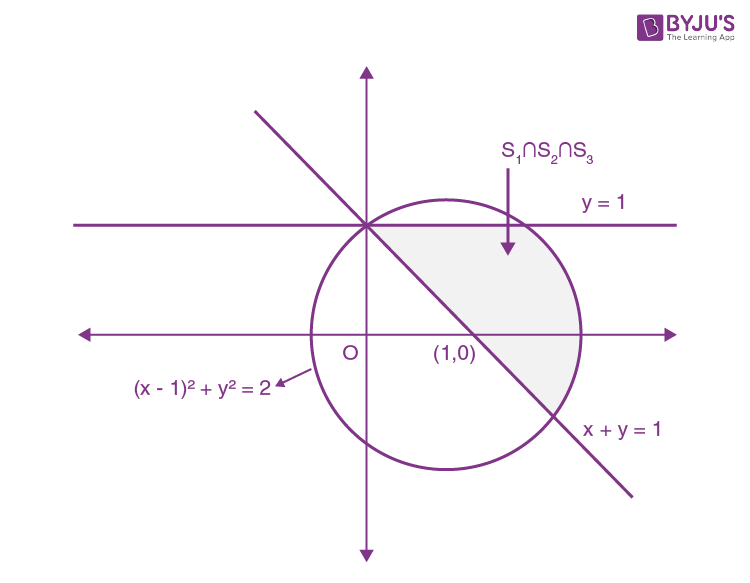Let, z = x + iy

S1 ≡ (x – 1)2 + y2 ≤ 2 …(1)

S2 ≡ x + y ≥ 1 …(2)

S3 ≡ y ≤ 1 …(3)

⇒ S1 ∩ S2 ∩ S3 has infinitely many elements.

Question 16: If the sides AB, BC, and CA of a triangle ABC have, 3, 5 and 6 interior points respectively, then the total number of triangles that can be constructed using these points as vertices is equal to:

a. 360
b. 240
c. 333
d. 364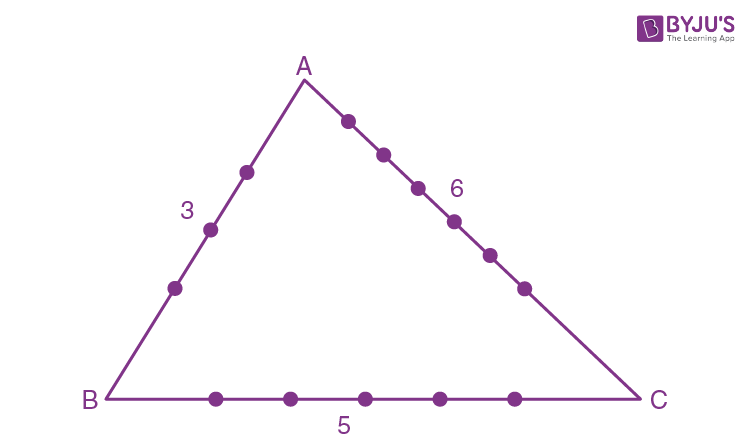Total number of triangles

= 3C1 × 5C1 × 6C1

+ 3C1 × 5C2 + 5C1 × 3C2

+ 3C1 × 6C2 + 6C1 × 3C2

+ 5C1 × 6C2 + 6C1 × 5C2

= 90 + 30 + 15 + 45 + 18 + 75 + 60 = 333

Question 17: The value of limx→∞ [r] + [2r] + ……. [nr] / [n2], where r is a non-zero real number and [r] denotes the greatest integer less than or equal to r, is equal to:

a. 0
b. r
c. r / 2
d. 2r

We know that

r ≤ [r] < r + 1 and

2r ≤ [2r] < 2r + 1

3r ≤ [3r] < 3r + 1

nr ≤ [nr] < nr + 1

______________________

r + 2r + ….. + nr ≤ [r] + [2r] + …..+ [nr] < (r + 2r + …..+ nr) + n

{[n (n + 1) / 2] * r / (n2)} ≤ [r] + [2r] + …… [nr] / n2 < {[n (n + 1) / 2] * r + (n) / (n2)}

Now,

limn→∞ [n (n + 1) . r / (2 . n2)] = r / 2

And limn→∞ {[n (n + 1) / 2] * r + (n) / (n2)} = r / 2

So, by Sandwich Theorem, we can conclude that limn→∞ [r] + [2r] + ……. [nr] / [n2] = r / 2

Question 18: The value of ∑r=06 (6Cr . 6C6-r) is equal to:

a. 1124
b. 924
c. 1324
d. 1024

r=06 (6Cr . 6C6-r) = 6C0.6C6 + 6C1.6C5 + ……. + 6C6.6C0

Now, (1 + x)6 (1 + x)6 = (6C0 + 6C1x + 6C2x2 + …. + 6C6x6) (6C0 + 6C1x + 6C2x2 + …. + 6C6x6)

Comparing coefficient of x6 both sides

6C0.6C6 + 6C1 +6C5 + ….. + 6C6.6C0= 12C6

= 924

Therefore, the correct answer is (2).

Question 19: Two tangents are drawn from a point P to the circle x2 + y2 – 2x – 4y + 4 = 0, such that the angle between these tangents is tan-1 (12/5), where tan-1 (12/5) ∈ (0, π). If the centre of the circle is denoted by C and these tangents touch the circle at points A and B, then the ratio of the areas of △PAB and △CAB is :

a. 11 : 4
b. 9 : 4
c. 2 : 1
d. 3 : 1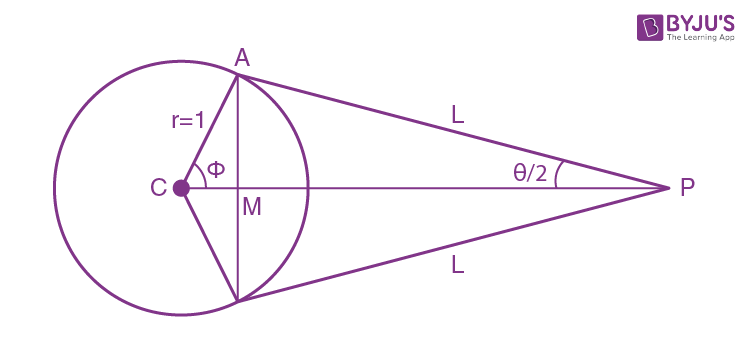Let θ = tan-1 (12 / 5)

⇒ tan θ = 12 / 5

⇒ [2 tan (θ / 2)] / [1 – tan2 (θ / 2)] = 12 / 5

⇒ tan (θ / 2) = 2 / 3 ⇒ sin (θ / 2) = 2 / √3 and cos (θ / 2) = 3 / √13

In ΔCAP, tan (θ / 2) = 1 / AP

⇒ AP = 3 / 2

In ΔAPM, sin (θ / 2) = AM / AP, cos (θ / 2) = AM / AP

⇒ AM = 3 / √13

⇒ PM = 9 / 2√13

∴ AB = 6 / √13

∴ Area of ΔPAB = (1 / 2) * AB * PM

(1 / 2) * (6 / √13) * (9 / 2√13) = 27 / 26

Now, φ = 90o – (θ / 2)

In ΔCAM,

cos φ = CM / CA

⇒ CM = 1 . cos [(π / 2) – (θ / 2)]

= 1 . sin (θ / 2) = 2 / √13

∴ Area of ΔCAB =(1 / 2) × AB × CM

= (1 / 2) * (6 / √13) * (2 / √13) = 6 / 13

∴ [Area of ΔPAB] / [Area of ΔCAB] = (27 / 26) / (6 / 13) = 9 / 4

Therefore, the correct answer is (2).

AliterArea of ΔPAB = RL3 / [R2 + L2] and Area of ΔCAB = LR3 / [R2 + L]

Therefore, required ratio = {RL3 / [R2 + L2]} / {LR3 / [R2 + L]} = (L / R)2

= cot2 (θ / 2) = [2 cos2 (θ / 2)] / [2 sin2 (θ / 2)]

= [1 + cos θ] / [1 – cos θ]

= [1 + (5 / 13)] / [1 – (5 / 13)]

= 9 / 4

Question 20: The number of solutions of the equation sin-1 [x2 + (1 / 3)] + cos-1 [x2 – (2 / 3)] = x2, for x ∈ [- 1, 1], and [x] denotes the greatest integer less than or equal to x, is:

a. 0
b. 2
c. 4
d. Infinite

There are three cases possible for x ∈ [– 1, 1]

Case I : x ∈ [- 1, – √(2/3) )

sin-1 (1) + cos-1 0 = x2

x2 = (π / 2) + (π / 2) = π ⇒ x = ± √π → (Reject)

Case II : x ∈ (- √(2/3), √(2/3) )

sin-1 (0) + cos-1 (- 1) = x2

0 + π = x2 ⇒ x = ± √π → (Reject)

Case III: x ∈ (- √(2/3), 1)

sin-1 (0) + cos-1 (0) = x2

π = x2 ⇒ x = ± √π → (Reject)

No solution exists.

Therefore, the correct answer is (1).

SECTION – B

Question 21: Let the coefficients of third, fourth and fifth terms in the expansion of [x + (a / x2)]n, x ≠ 0 be in the ratio 12:8:3. Then the term independent of x in the expansion is equal to ………

Tr+1 = ncr xn-r (a / x2)r = ncr ar xn-3r

T3 = nc2 a2 xn-6, T4 = nc3 a3 xn-9

T5 = nc4 a4 xn-12

Now, [coefficient of T3] / [coefficient of T4] = [nc2 a2] / [nc3 a3] = 3 / [a (n – 2)] = 3 / 2

a(n – 2) = 2 ……… (i) and [coefficient of T4] / [coefficient of T5] = [nc3 a3] / [nc4 a4] = 4 / [a (n – 3)] = 8 / 3

a(n – 3) = 3 / 2 ……… (ii)

By (i) and (ii) n = 6, a = 1 / 2

For term independent of ‘x’,

n – 3r = 0

r = n / 3

r = 6 / 3 = 2

T3 = 6c2 (1 / 2)2 x0 = 15 / 4 = 3.75 ≅ 4

Question 22: Let A =

$$\begin{array}{l}\begin{bmatrix} a &b \\ c&d \end{bmatrix}\end{array}$$
and B=
$$\begin{array}{l}\begin{bmatrix} \alpha \\ \beta \end{bmatrix}\neq \begin{bmatrix} 0\\ 0 \end{bmatrix}\end{array}$$
such that AB = B and a + d = 2021, then the value of ad-bc is equal to …………….

$$\begin{array}{l}\\A=\begin{bmatrix} a &b \\ c&d \end{bmatrix}\\B=\begin{bmatrix} \alpha \\ \beta \end{bmatrix}\\ AB=B\\ \begin{bmatrix} a &b \\ c&d \end{bmatrix}\begin{bmatrix} \alpha \\ \beta \end{bmatrix}=\begin{bmatrix} \alpha \\ \beta \end{bmatrix}\\ a\alpha +b\beta =\alpha —(1)\\ c\alpha +d\beta =\beta —-(2)\\\end{array}$$

ɑ (a – 1) = – bβ and cɑ = β (1 – d)

(ɑ / β) = – b / (a – 1) and (ɑ / β) = (1 – d) / c

– b / (a – 1) = (1 – d) / c

–bc = (a – 1) (1 – d)

–bc = a – ad – 1 + d

ad – bc = a + d – 1

= 2021–1

= 2020

Question 23: Let f : [–1,1] → R be defined as f (x) = ax2 + bx + c for all x ∈ [–1, 1], where a, b, c ∈ R such that f (–1) = 2, f’ (–1) = 1 and for x ∈ [–1, 1] the maximum value of f’’ (x) is 1 / 2. If f (x) ≤ ɑ, x ∈ [–1, 1], then the least value of ɑ is equal to …………… .

f (x) = ax2 + bx + c

f’ (x) = 2ax + b,

f” (x) = 2a

Given f” (–1) = 1 / 2 ⇒ a =1 / 4

f’ (–1) = 1 ⇒ b – 2a = 1 ⇒ b = 3 /2

f (–1) = a – b + c = 2 ⇒ c = 13 / 4

Now f (x) = (1/4) (x2 + 6x + 13), x ∈ [–1, 1]

f’ (x) = (1/4) (2x + 6) = 0 ⇒ x = –3 ∉ [–1, 1]

f (1) = 5, f (–1) = 2

f (x) ≤ 5

So αminimum = 5

Question 24: Let In = ∫1e x19 (log |x|)n dx, where n ∈ N. If 20 (I10) = αI9 + βI8, for natural numbers α and β, then α – β equal to ………………… .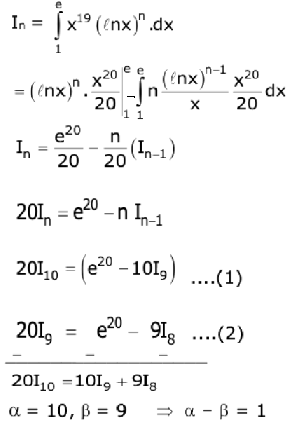Question 25: Let f : [–3, 1]→ R be given as

$$\begin{array}{l}f(x)=\left\{\begin{matrix} min[(x+6,x^{2})], &-3\leq x\leq 0 \\ max[\sqrt{x},x^{2}],&0\leq x\leq 1 \end{matrix}\right.\end{array}$$
If the area bounded by y = f(x) and x-axis is A, then the value of 6A is equal to …………… .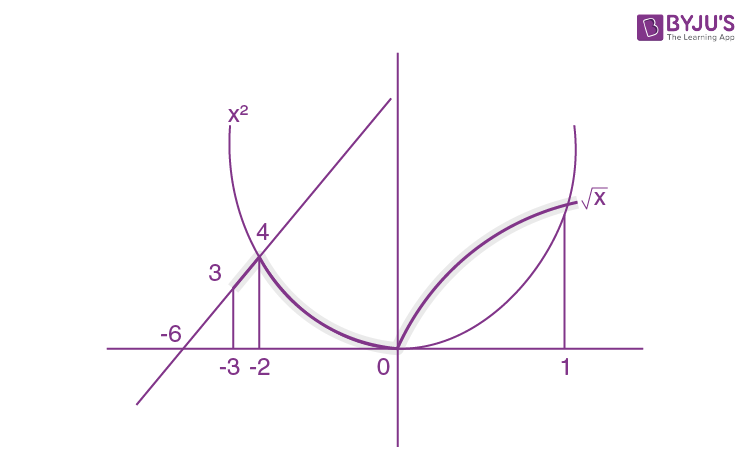Area is ∫-3-2 (x + 6) dx + ∫-20 x2 dx + ∫01 √x dx = A

= (7 / 2) + (x3 / 3)-20 + [(2 / 3) x3/2]01

= (7 / 2) + (8 / 3) + (2 / 3)

= 41 / 6

So, 6A = 41

Question 26: Let x be a vector in the plane containing vectors a = 2i – j + k and b = i + 2j – k. If the vector x is perpendicular to (3i + 2j – k) and its projection on a is 17√6 / 2 , then the value of |x|2 is equal to ……………….. .

Let x = k (a + λb)

I. The vector x is perpendicular to 3i + 2j – k.

k{(2 + λ)3 + (2λ – 1)2 + (1 – λ)(–1) = 0

⇒ 8λ + 3 = 0

λ = – 3 / 8

II. Also projection of x on a is therefore

[x . a] / |a| = 17√6 / 2

⇒ k [(a + λb) . a] / √6 = 17√6 / 2

⇒ k [6 + (3 / 8)] = [17 * 6] / 2

⇒ k = (51 / 51) * 8

k = 8

x = 8 [(13 / 8) i – (14 / 8) j + (11 / 8) k]

= 13i – 14j + 11k

|x|2 = 169 + 196 + 121

= 486

Question 27: Consider a set of 3n numbers having variance 4. In this set, the mean of the first 2n numbers is 6 and the mean of the remaining n numbers is 3. A new set is constructed by adding 1 into each of the first 2n numbers and subtracting 1 from each of the remaining n numbers. If the variance of the new set is k, then 9k is equal to ………………..

Let first 2n observations are x1, x2 ………… , x2n and last n observations are y1, y2 ………… , yn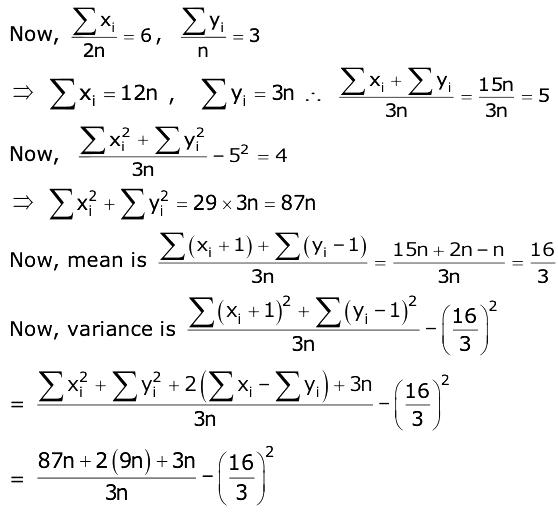= 29 + 6 + 1 – (16 / 3)2

= [324 – 256] / 9

= 68 / 9 = k

9k = 68

Therefore, the correct answer is 68.

Question 28: If 1, log10 (4x – 2) and log10 [4x + (18 / 5)] are in arithmetic progression for a real number x, then the value of the determinant

$$\begin{array}{l}\begin{vmatrix} 2[x-(1/2)] &x-1 &x^{2} \\ 1&0 &x \\ x&1 &0 \end{vmatrix}\end{array}$$
is equal to:

1, log10 (4x – 2) and log10 [4x + (18 / 5)] are in AP.

2. log10 (4x – 2) = 1 + log10 [4x + (18 / 5)]

log10 [4x – 2]2 = log10 [10 . [4x + (18 / 5)] [4x – 2]2 = 10 . [4x + (18 / 5)]

(4x)2 + 4 – 4 . 4x = 10 . 4x + 36

(4x)2 – 14 . 4x – 32 = 0

(4x)2 + 2 . 4x – 16 . 4x – 32 = 0

4x [4x + 2] – 16 [4x + 2] = 0

4x = – 2 or 4x = 16

Rejected the value of 4x = – 2.

Therefore

$$\begin{array}{l}\begin{vmatrix} 2[x-(1/2)] &x-1 &x^{2} \\ 1&0 &x \\ x&1 &0 \end{vmatrix}\end{array}$$

=

$$\begin{array}{l}\begin{vmatrix} 3 &1 &4 \\ 1&0 &2 \\ 2&1 &0 \end{vmatrix}\end{array}$$

= 3 (–2) – 1 (0 – 4) + 4 (1 – 0)

= – 6 + 4 + 4

= 2

Question 29: Let P be an arbitrary point having the sum of the squares of the distances from the planes x + y + z = 0, lx – nz = 0 and x – 2y + z = 0, equal to 9. If the locus of the point P is x2 + y2 +z2 = 9, then the value of l – n is equal to ………………

Let point P is (α, β, γ)

{[α + β + γ] / √3}2 + {[lα – nγ] / (√l2 + n2)]2 + {[α – 2β + γ)] / [√6]}2 = 9

Locus is [(x + y + z)2 / 3] + [(ln – nz)2 / (l2 + n2)] + [(x – 2y + z)2 / 6] = 9

Since its given that x2 + y2 + z2 = 9

After solving l = n, then l – n = 0

Question 30: Let tan α, tan β and tan γ; α, β, γ ≠ [2n – 1] π / 2, n ∈ N be the slopes of three-line segment OA, OB and OC, respectively, where O is the origin. If the circumcentre of triangle ABC coincides with the origin and its orthocentre lies on the y-axis, then the value of {[cos 3α + cos 3β + cos 3γ] / [cos α * cos β * cos γ]}2 is equal to

Since orthocentre and circumcentre both lies on y-axis

Centroid also lies on y-axis

Σcos α = 0

cosα + cos β + cosγ = 0

cos3α + cos3β + cos3γ = 3 cos α * cos β * cos γ

{[cos 3α + cos 3β + cos 3γ] / [cos α * cos β * cos γ]}

= {4 ([cos3α + cos3β + cos3γ] – 3 [cos α + cos β + cos γ]} / [cos α * cos β * cos γ] = 12

Then, {[cos 3α + cos 3β + cos 3γ] / [cos α * cos β * cos γ]}2 = 144